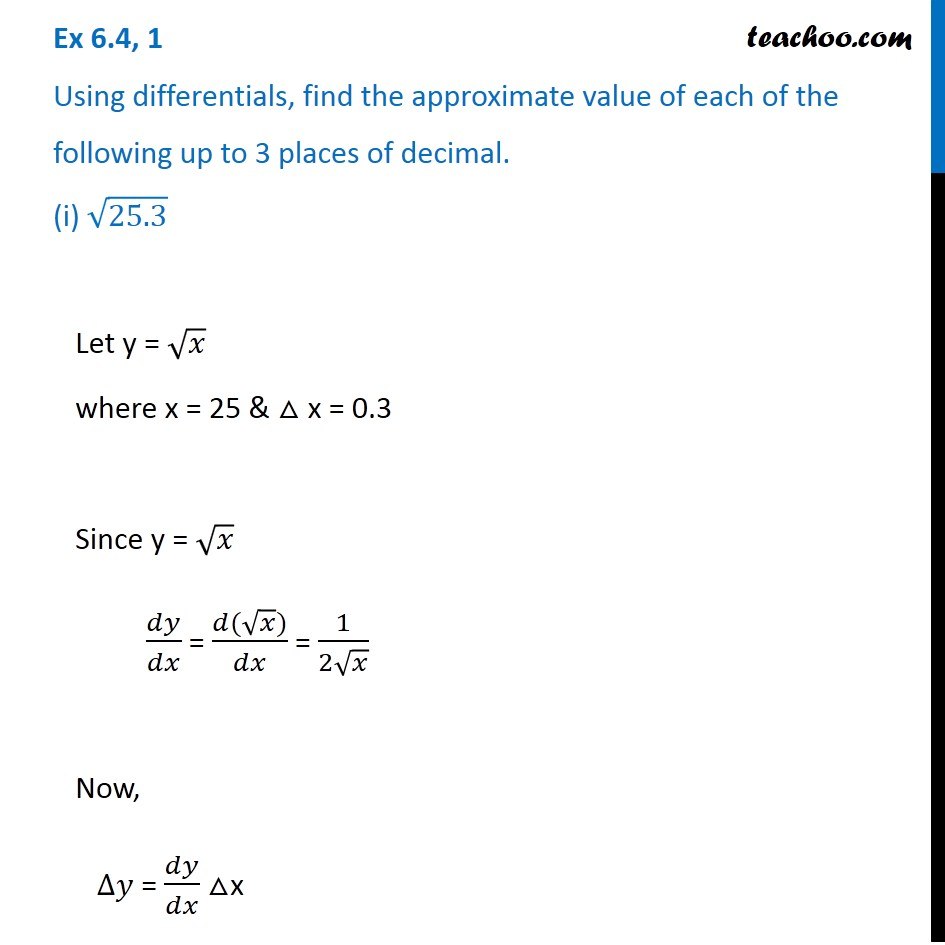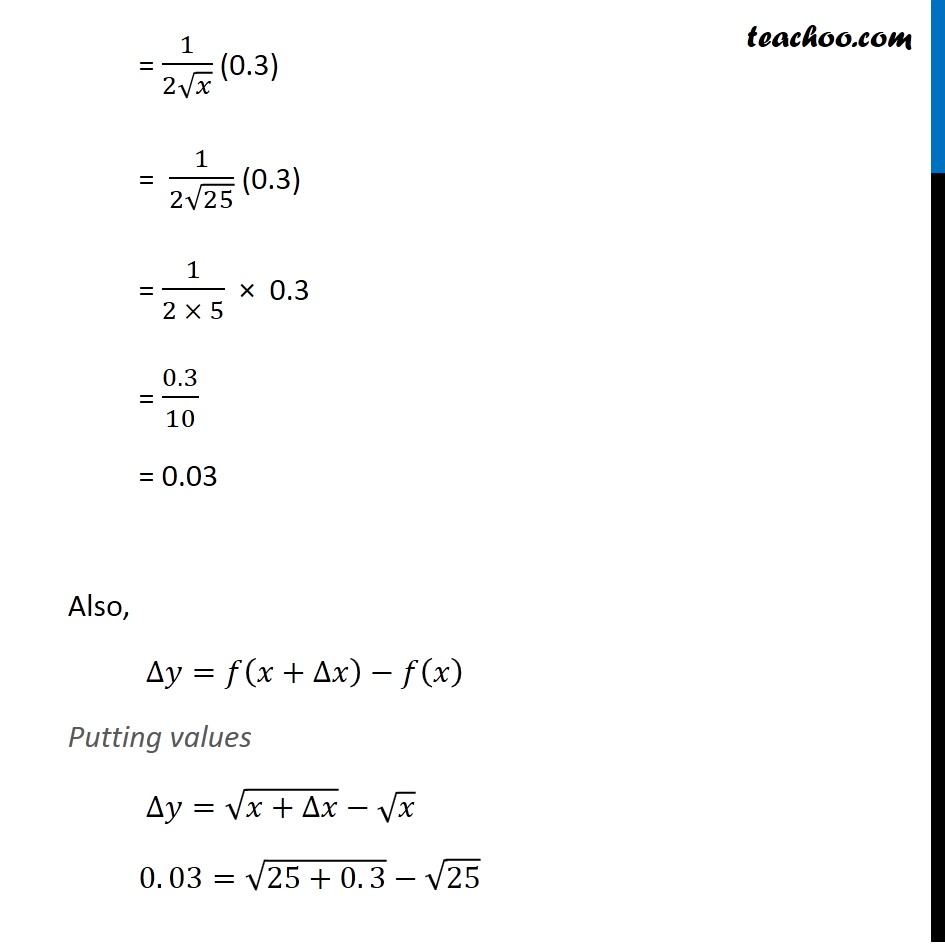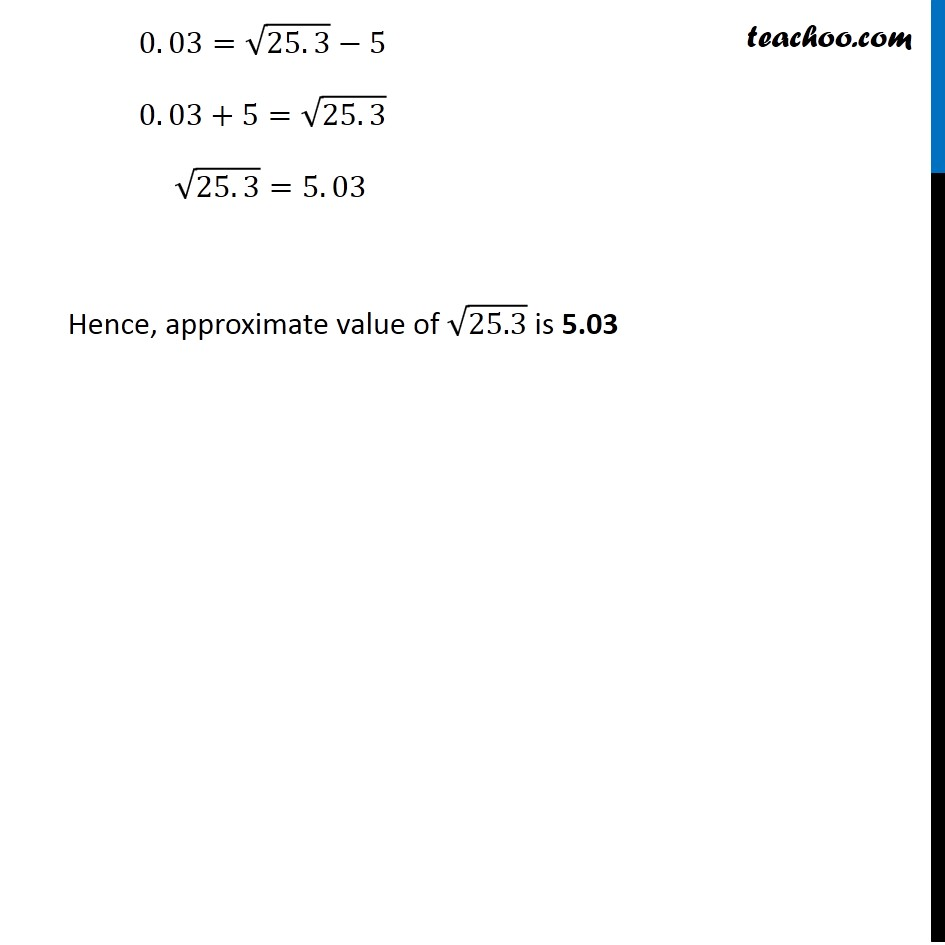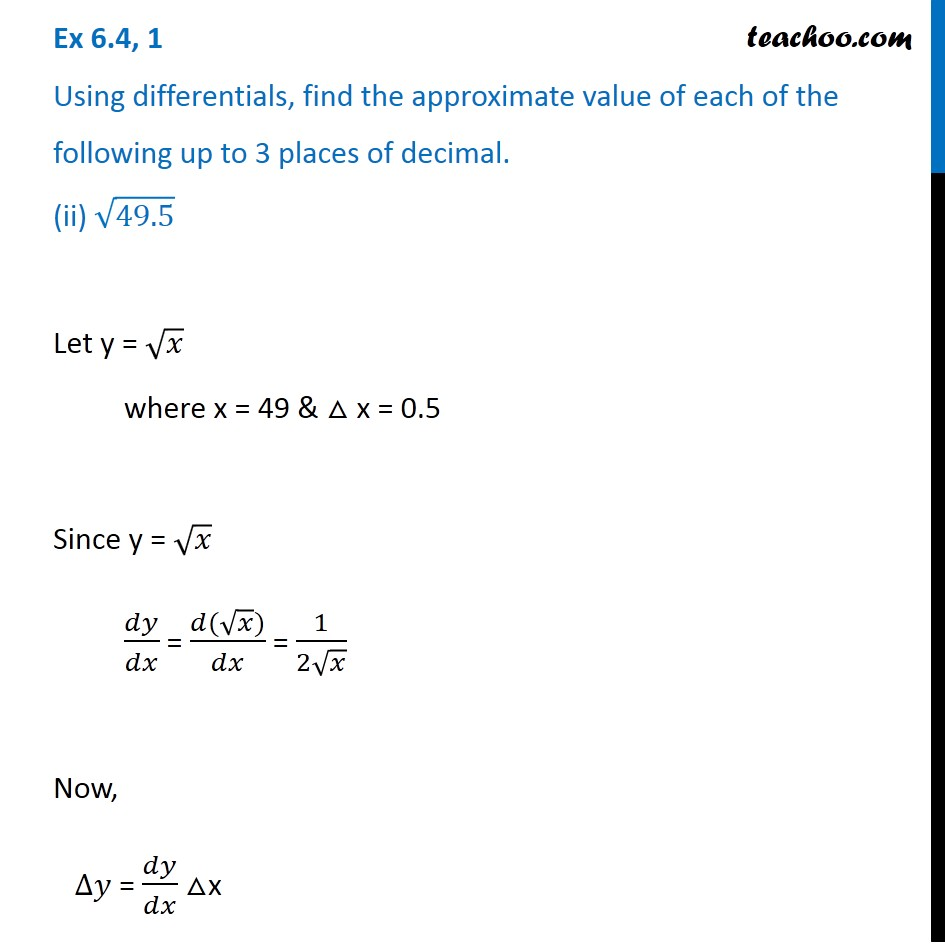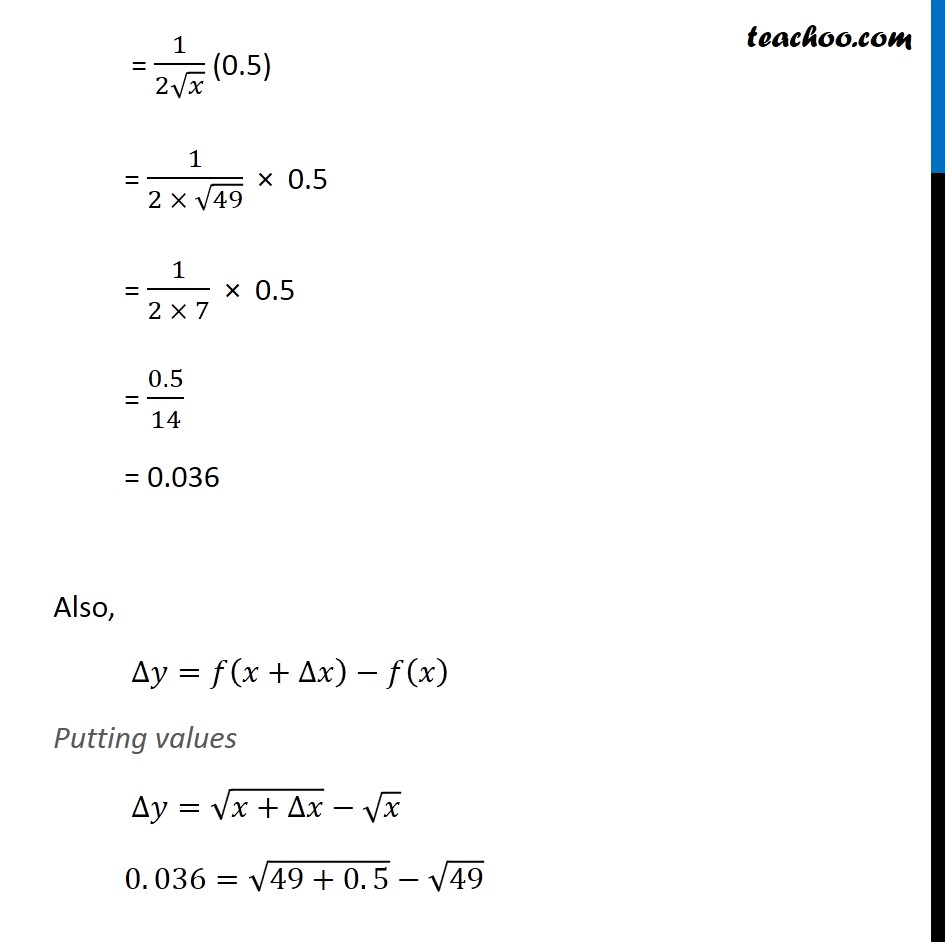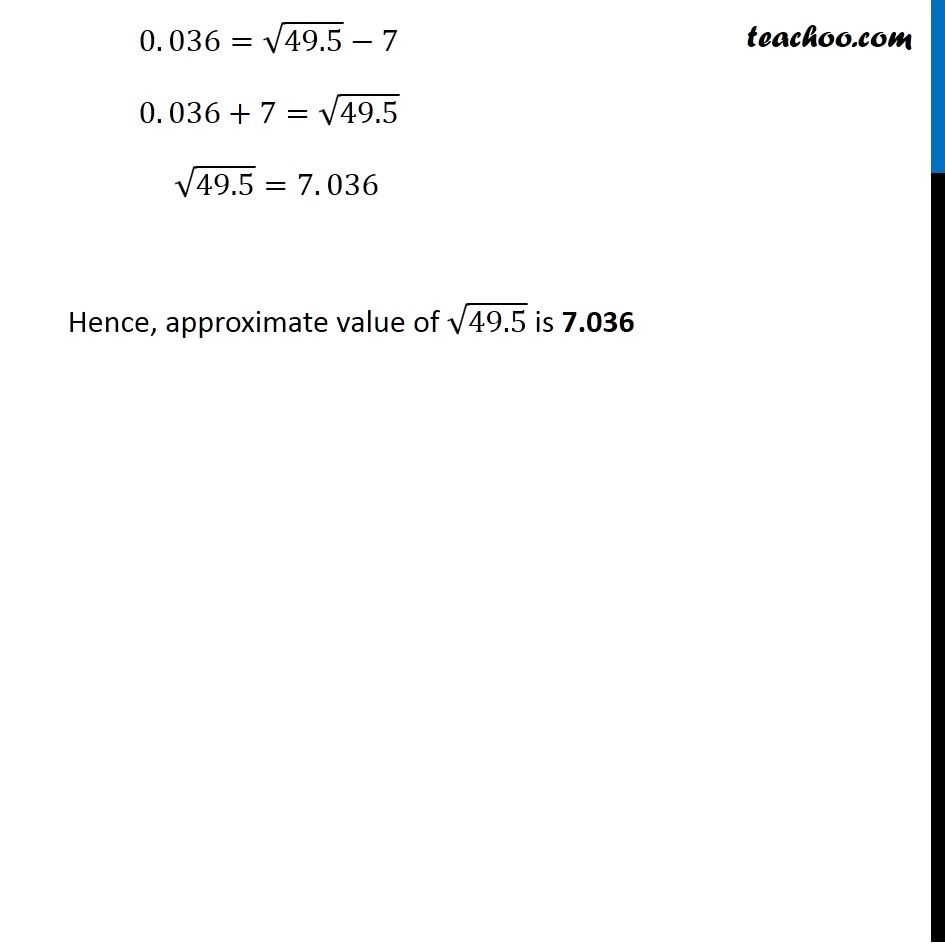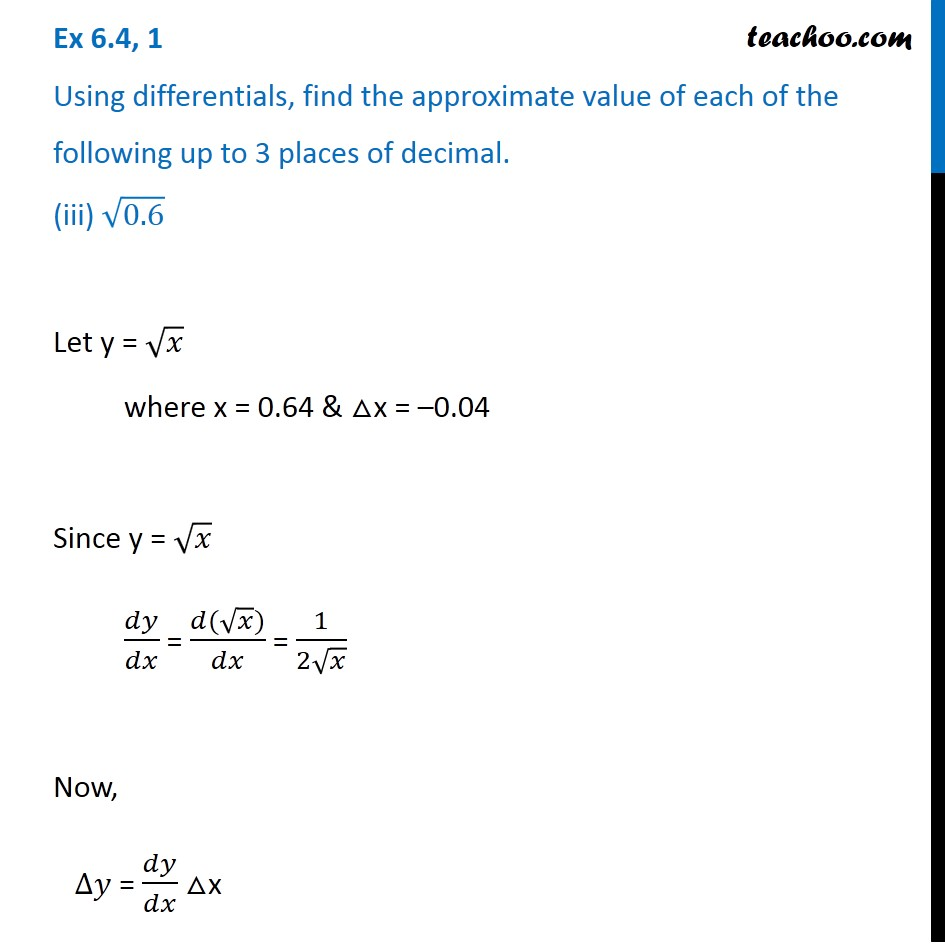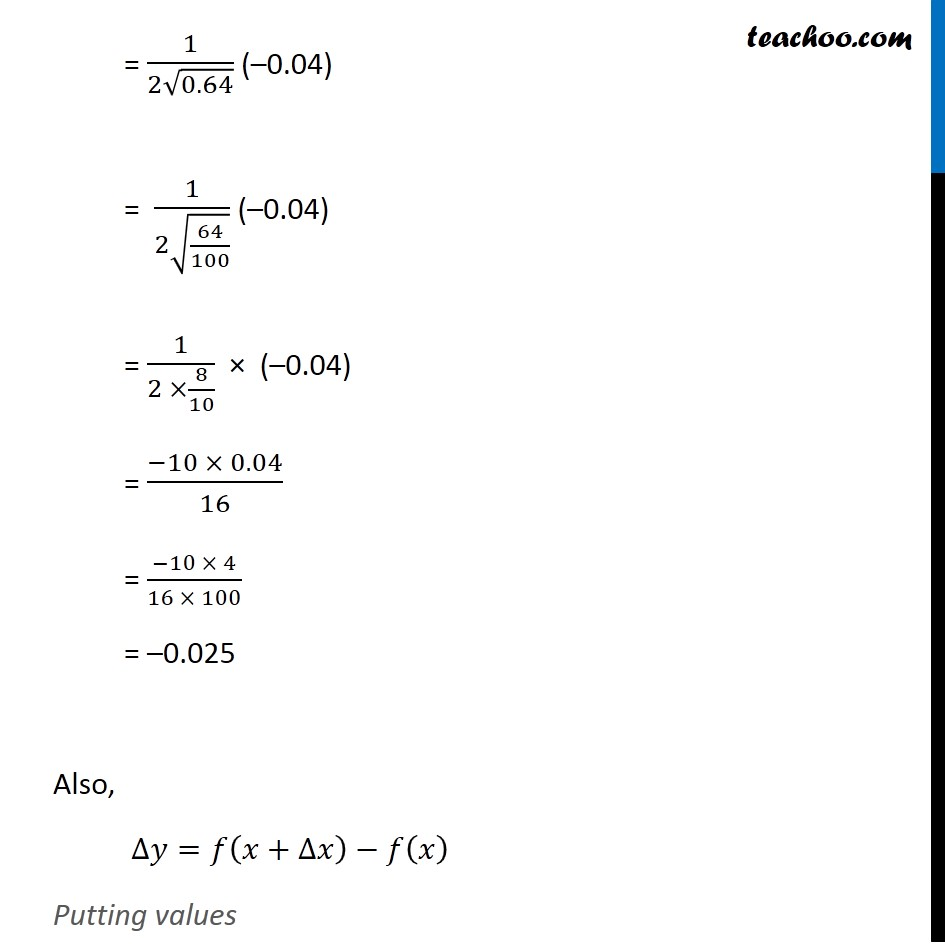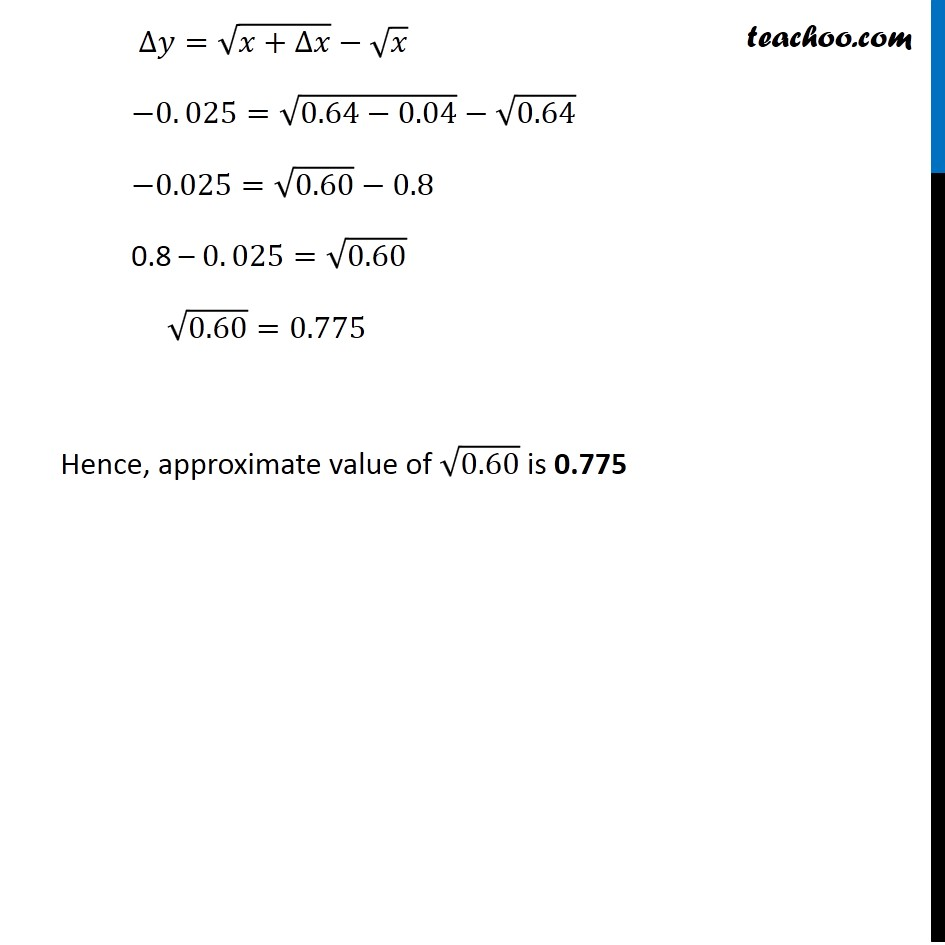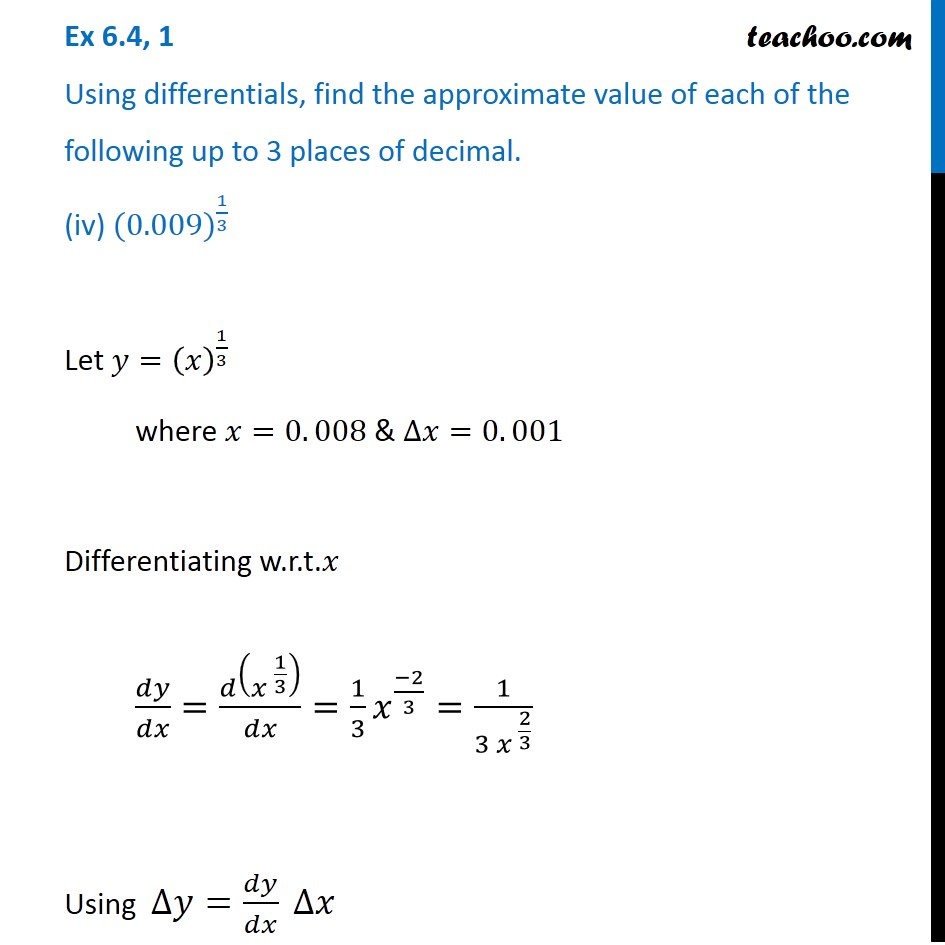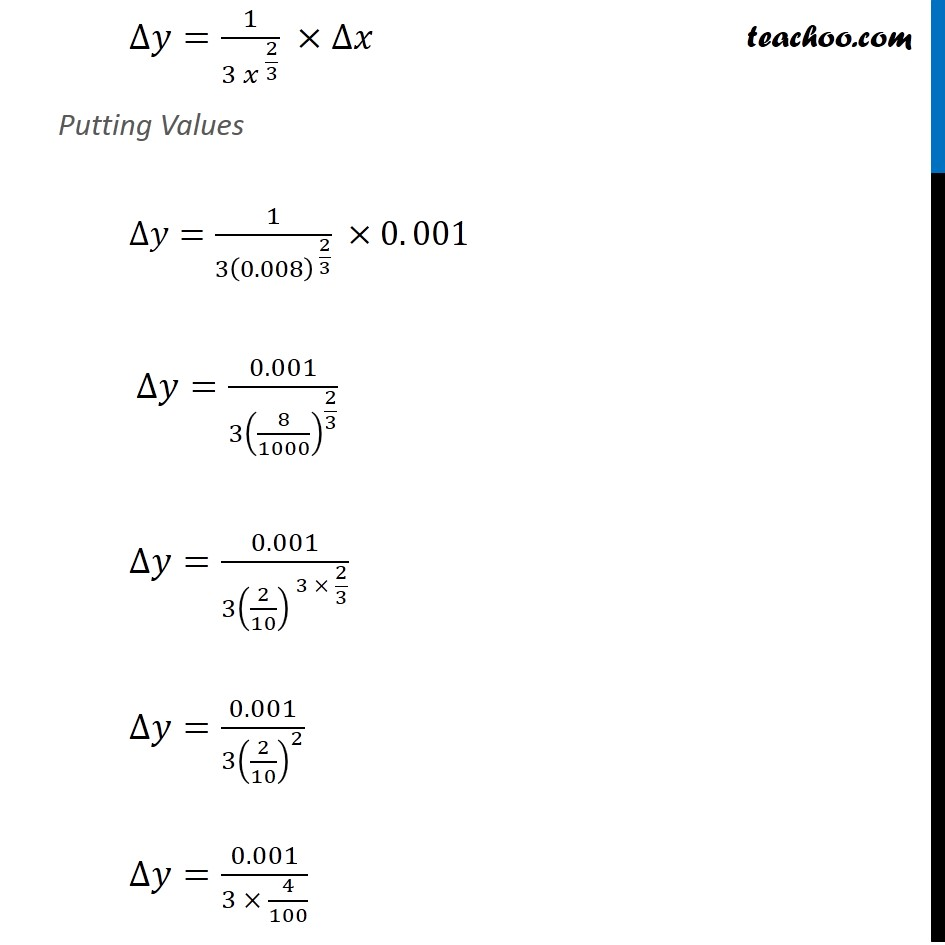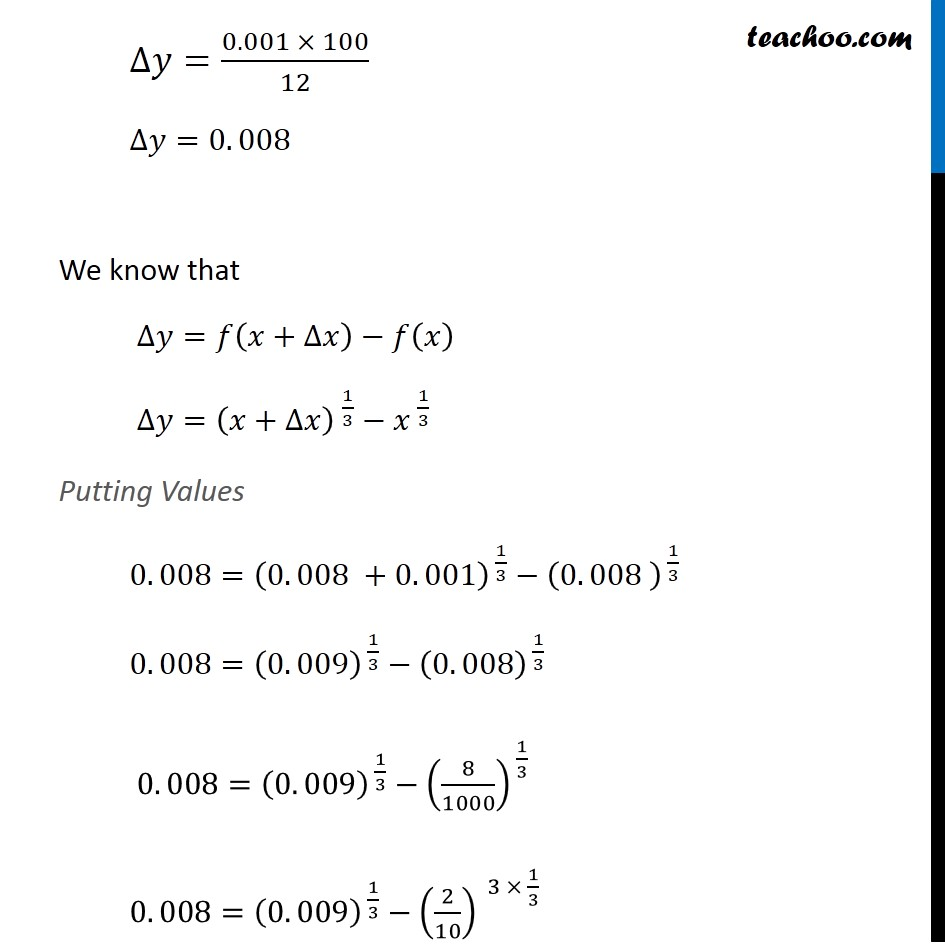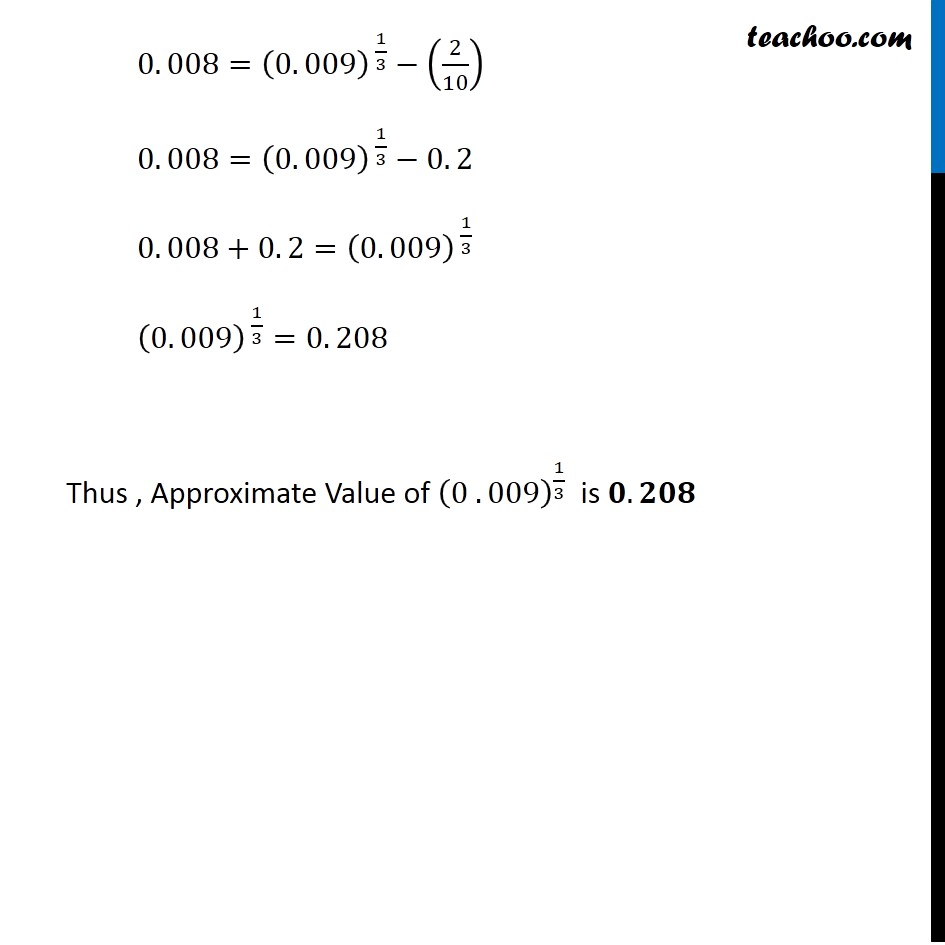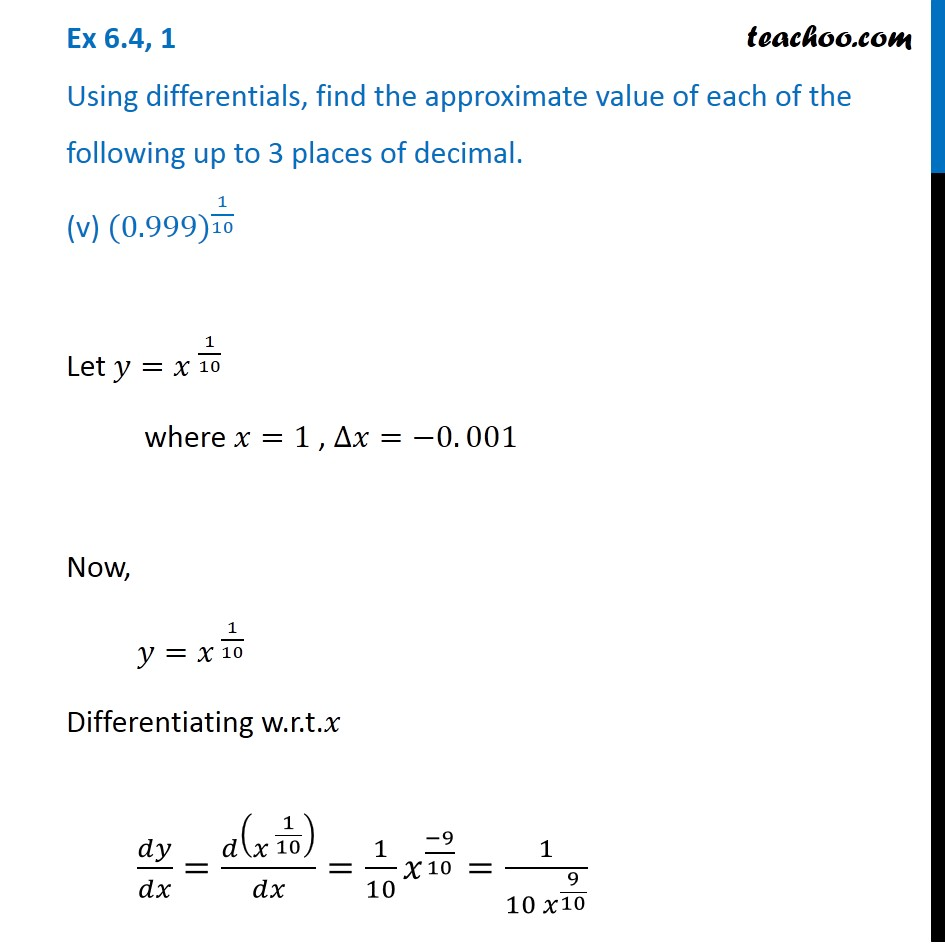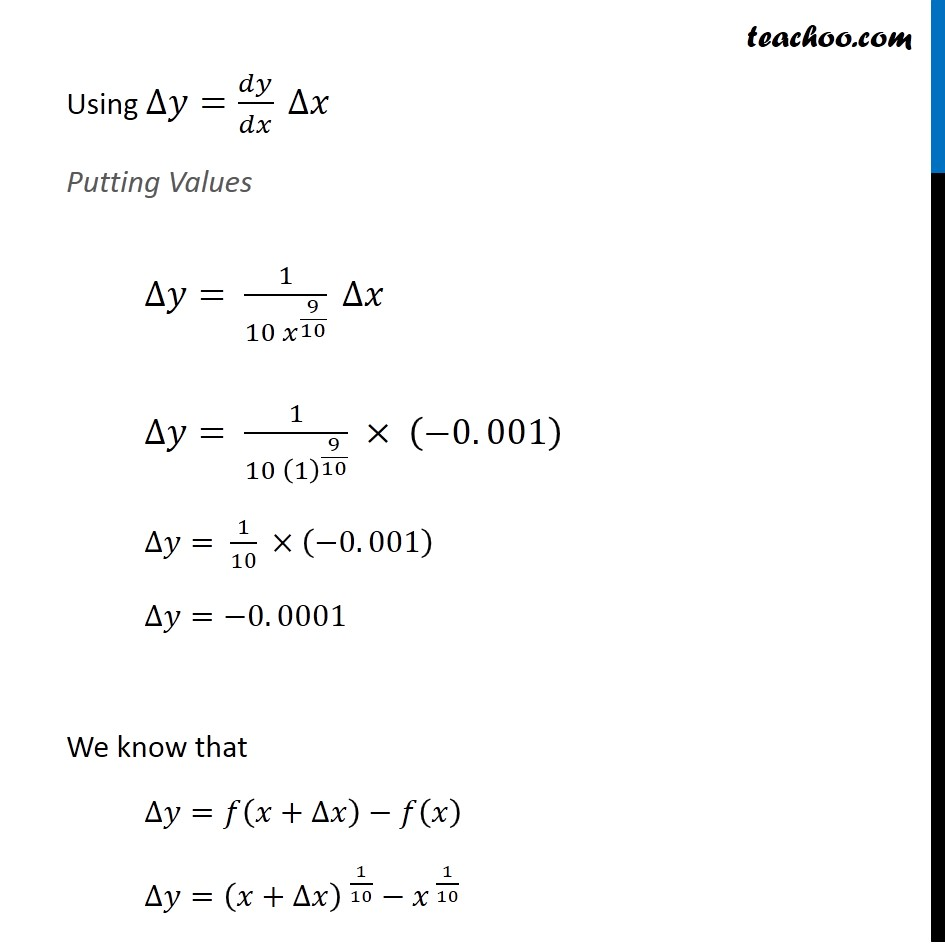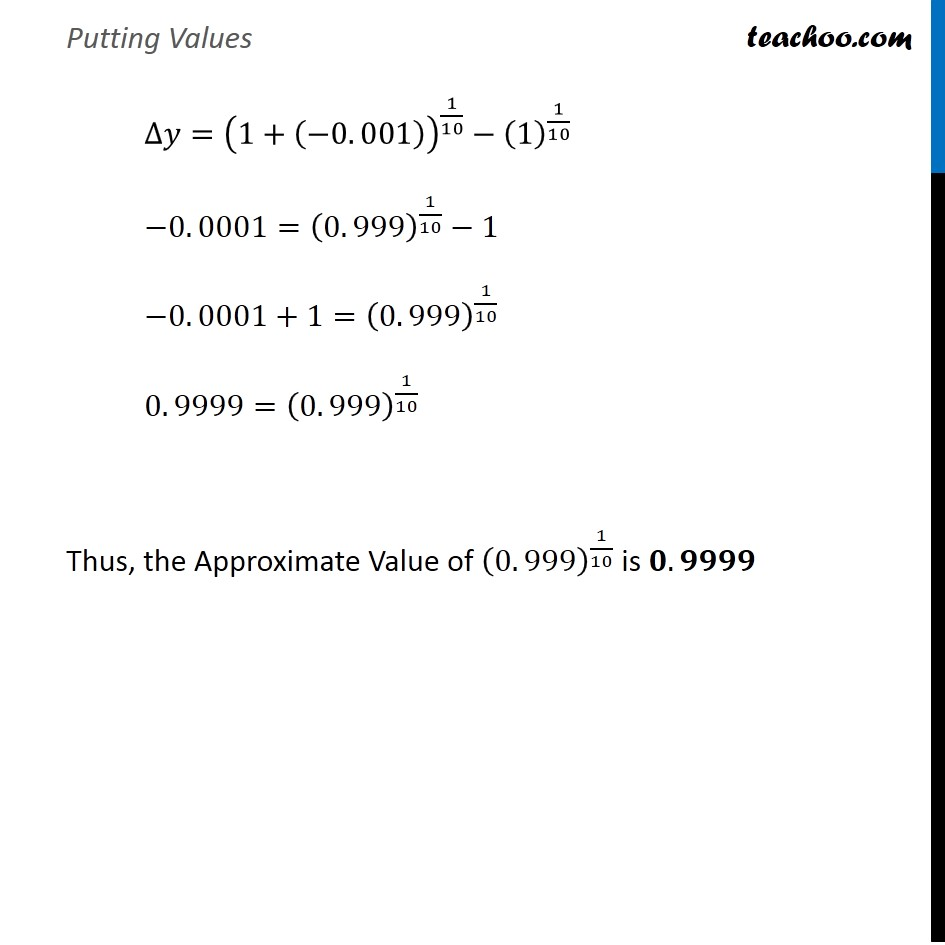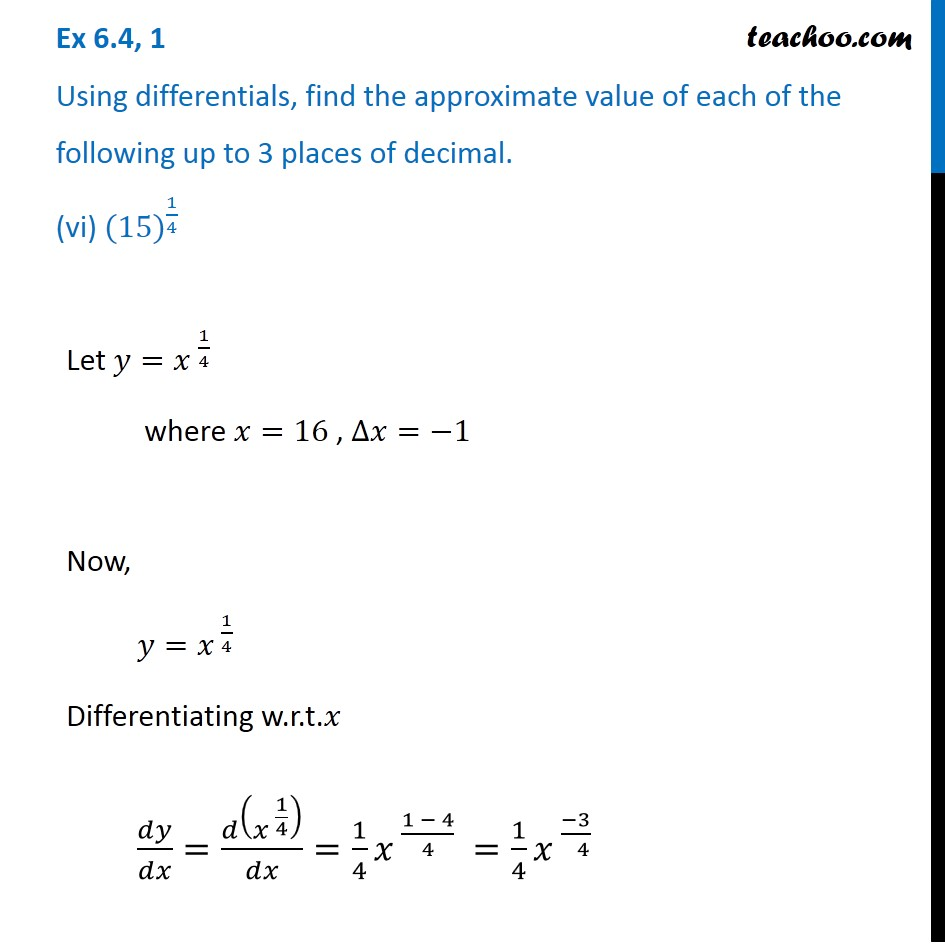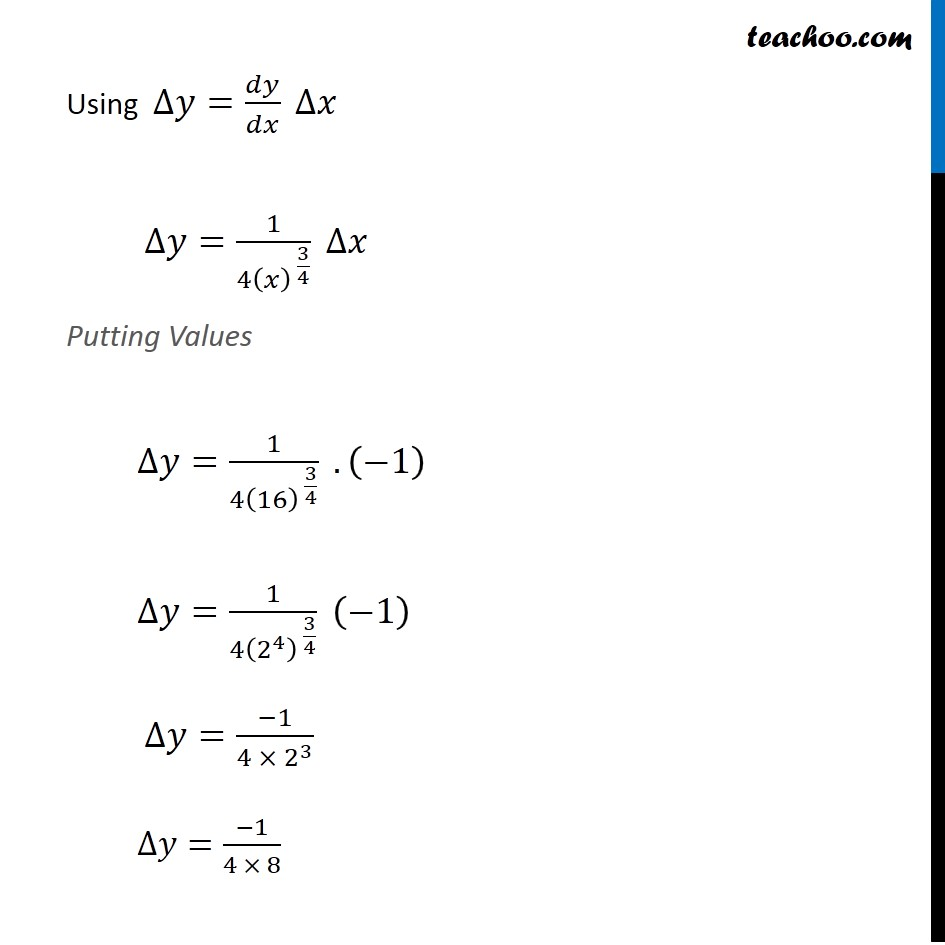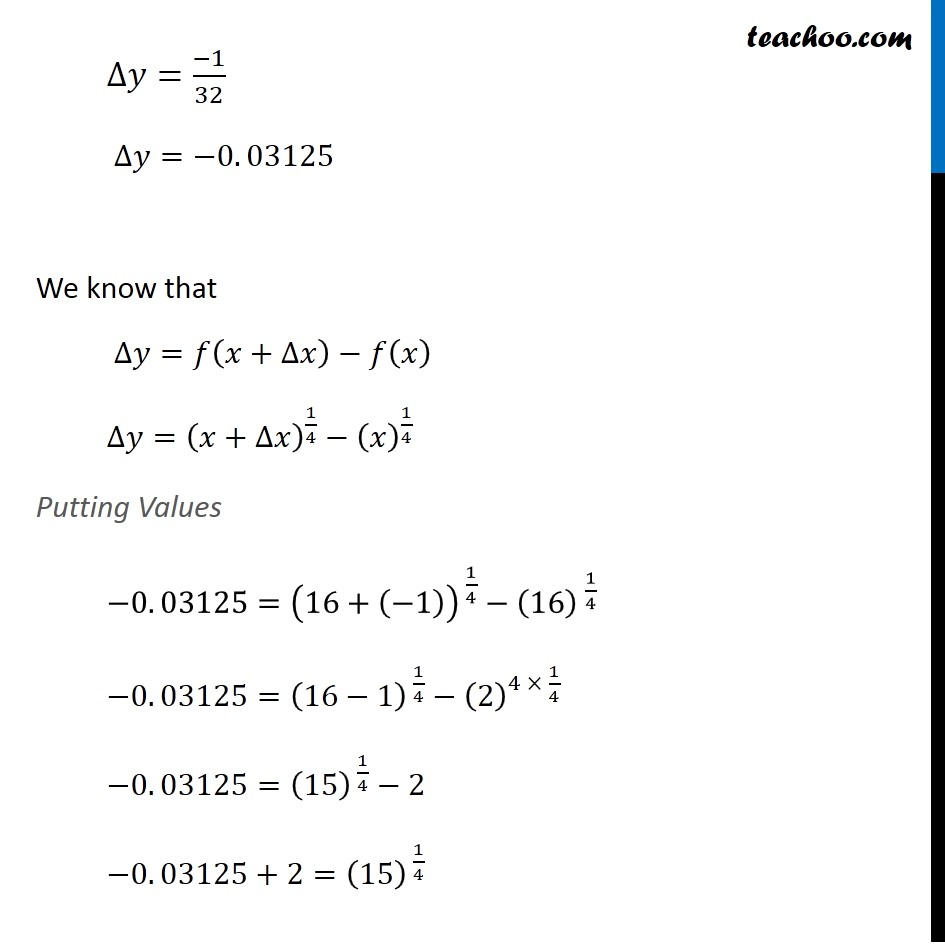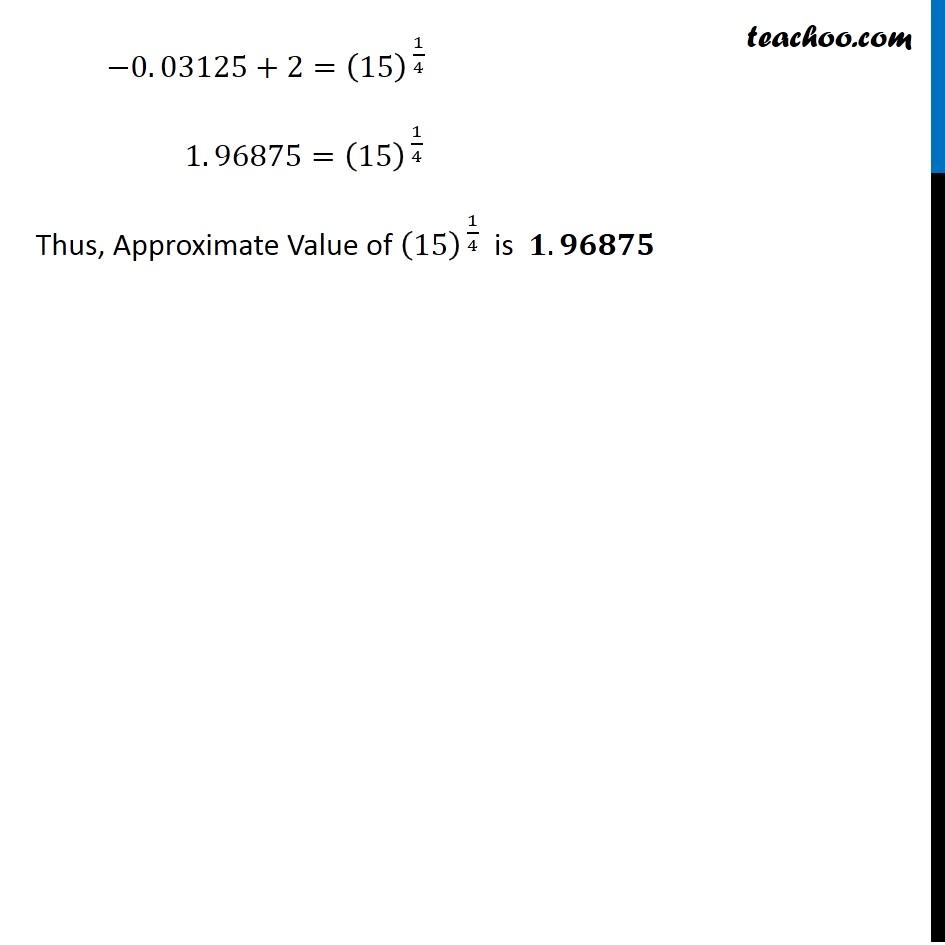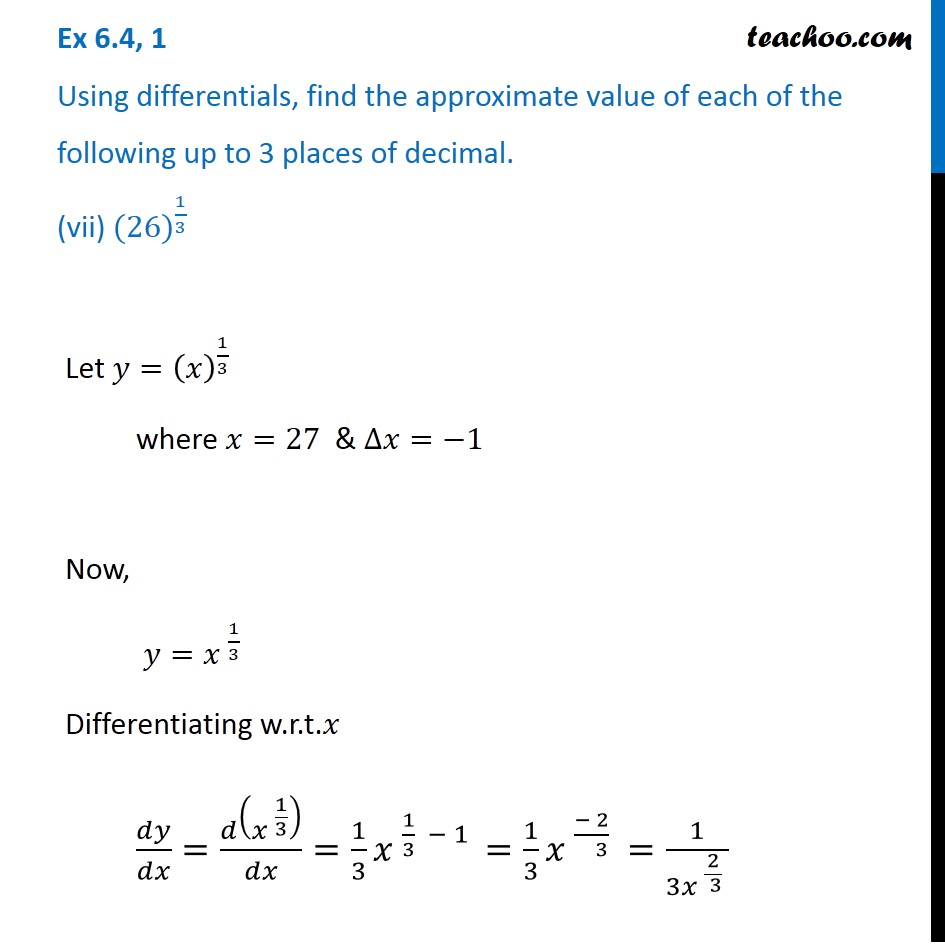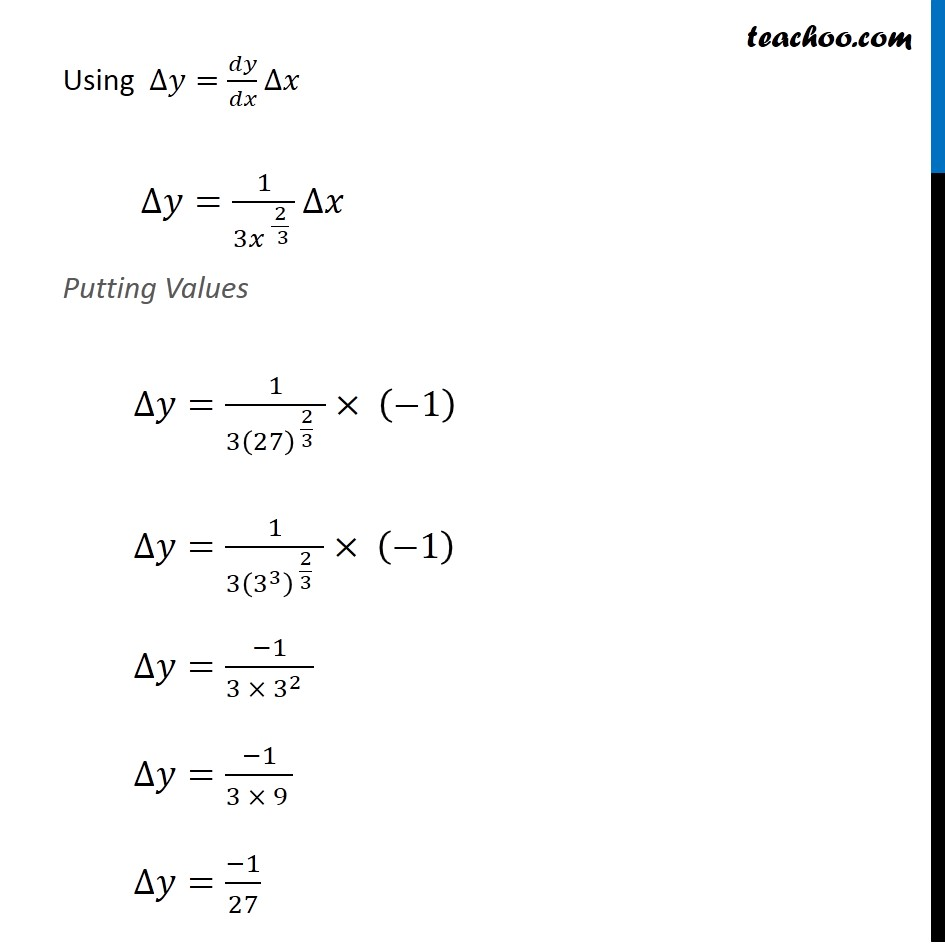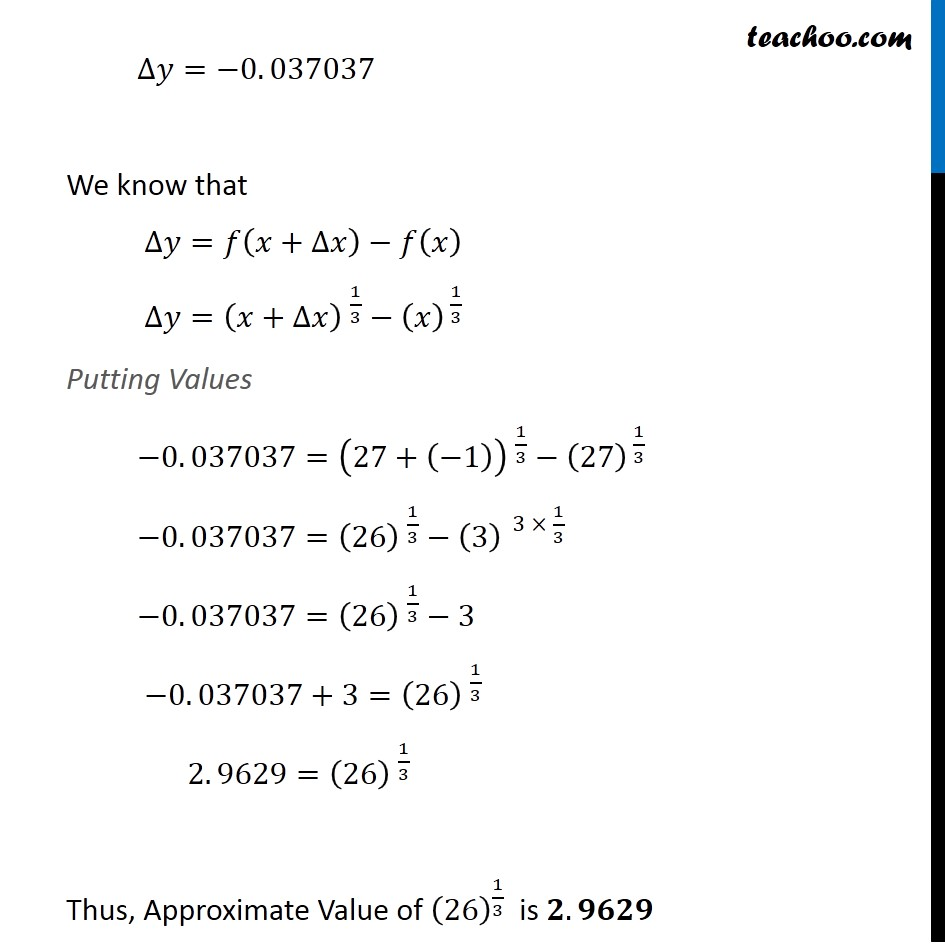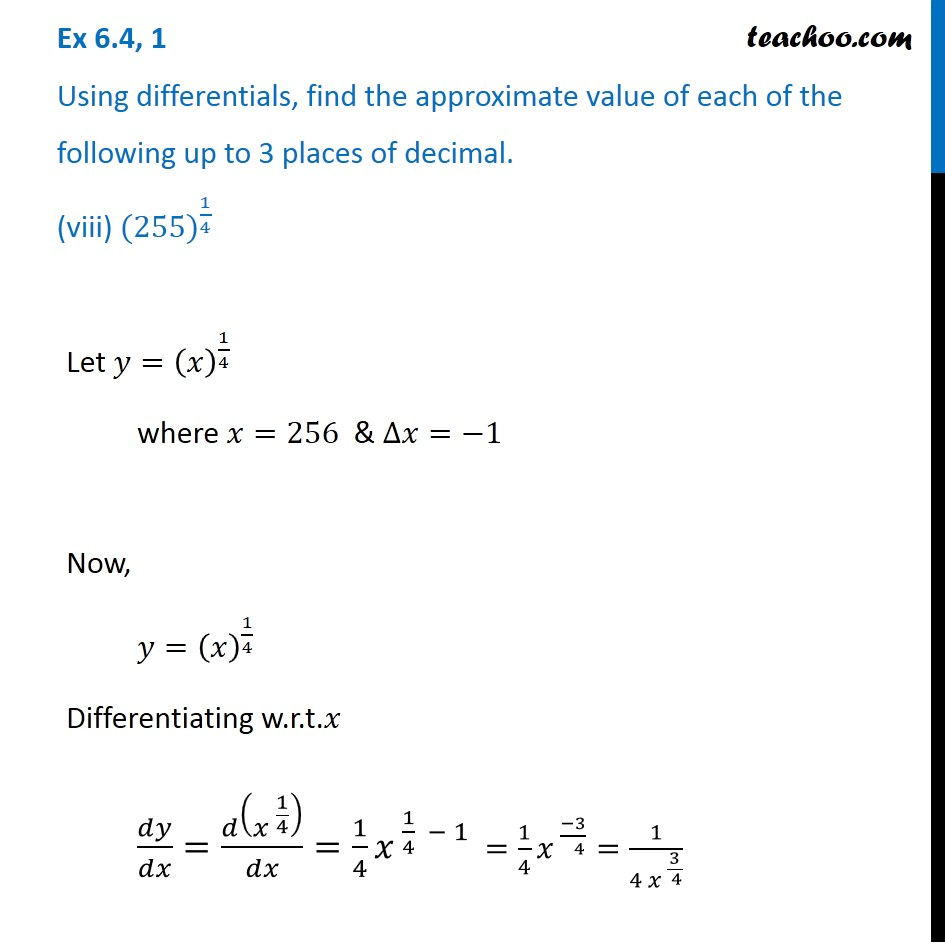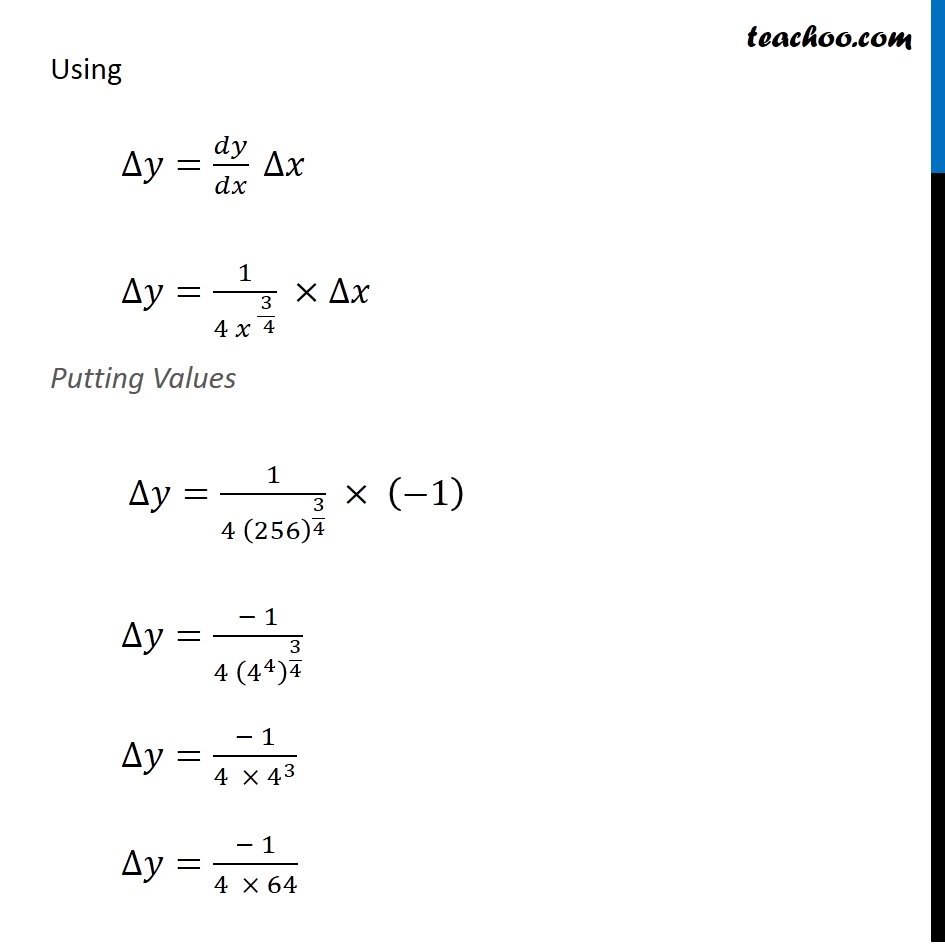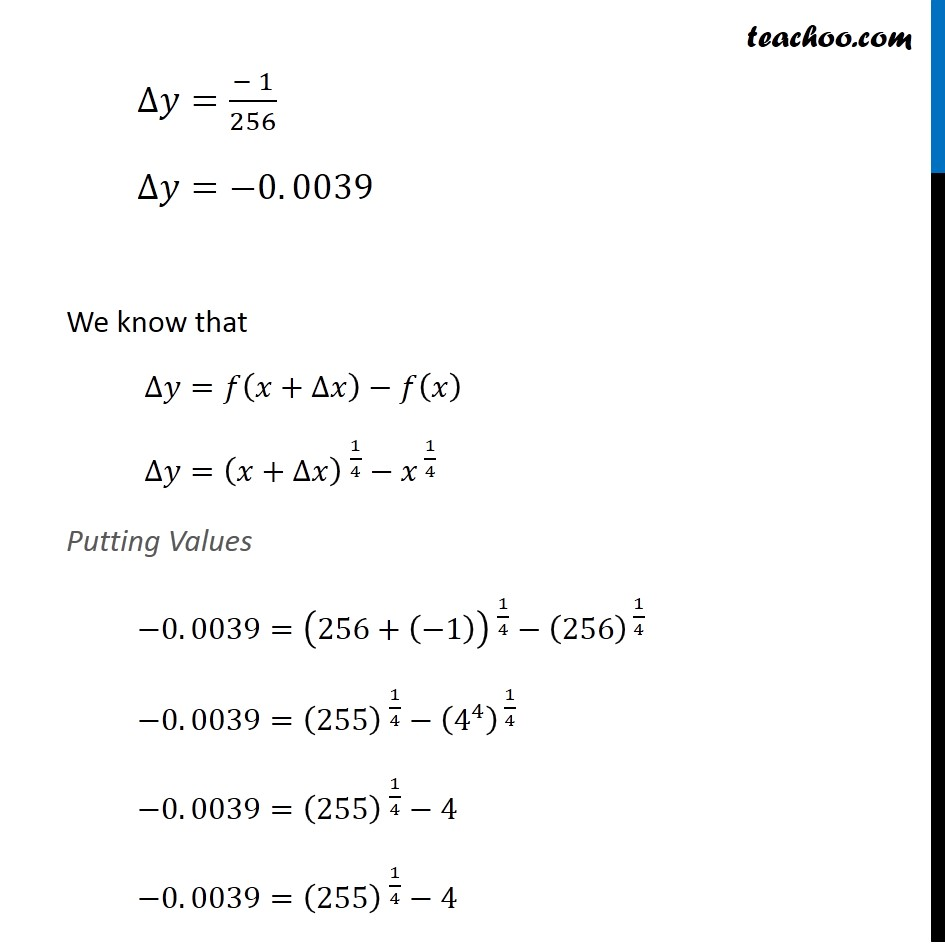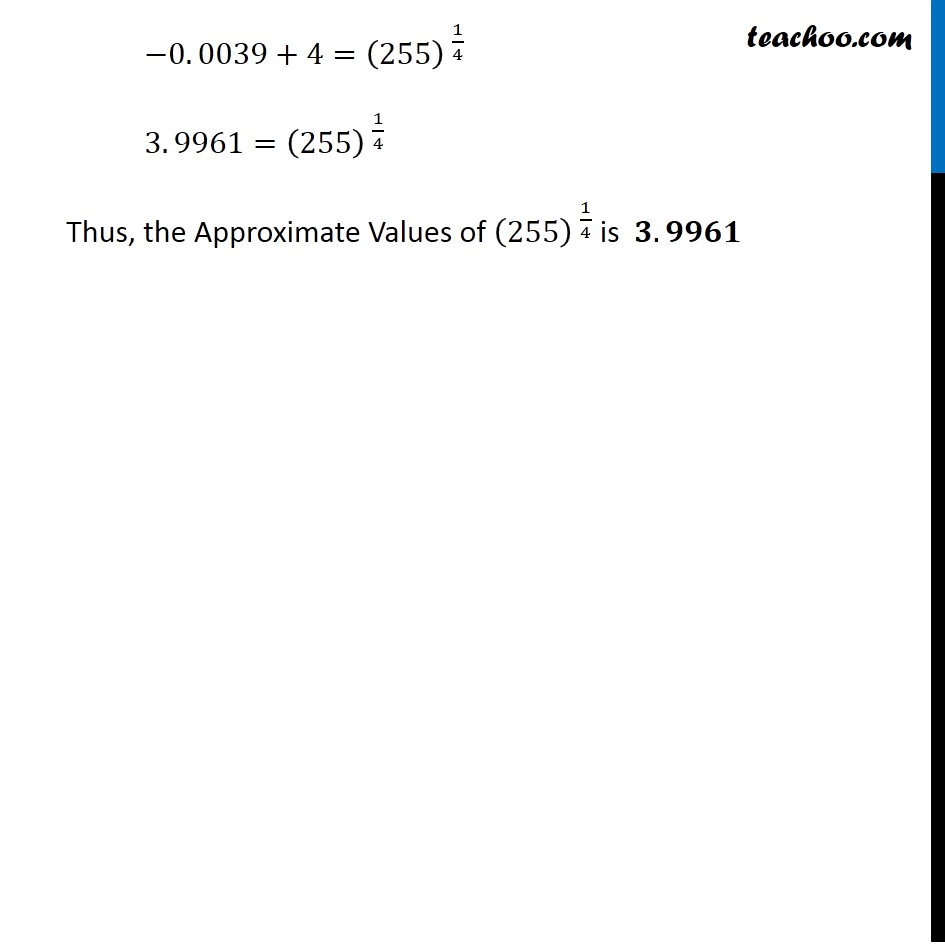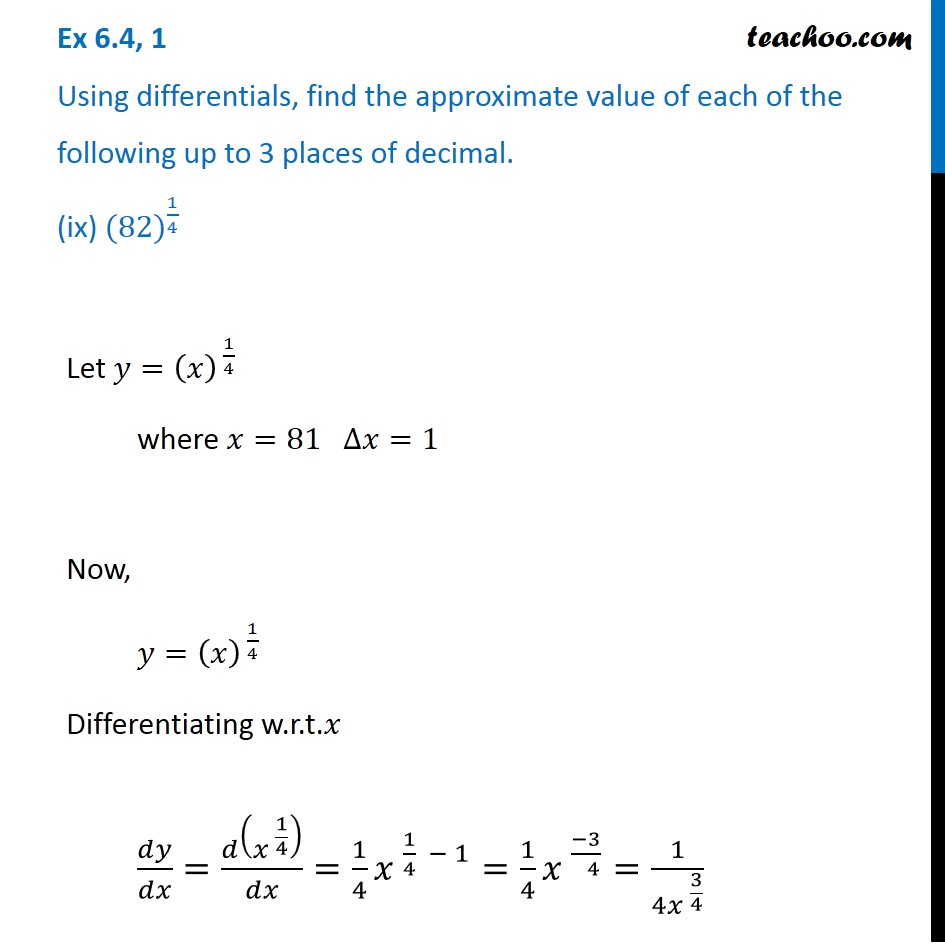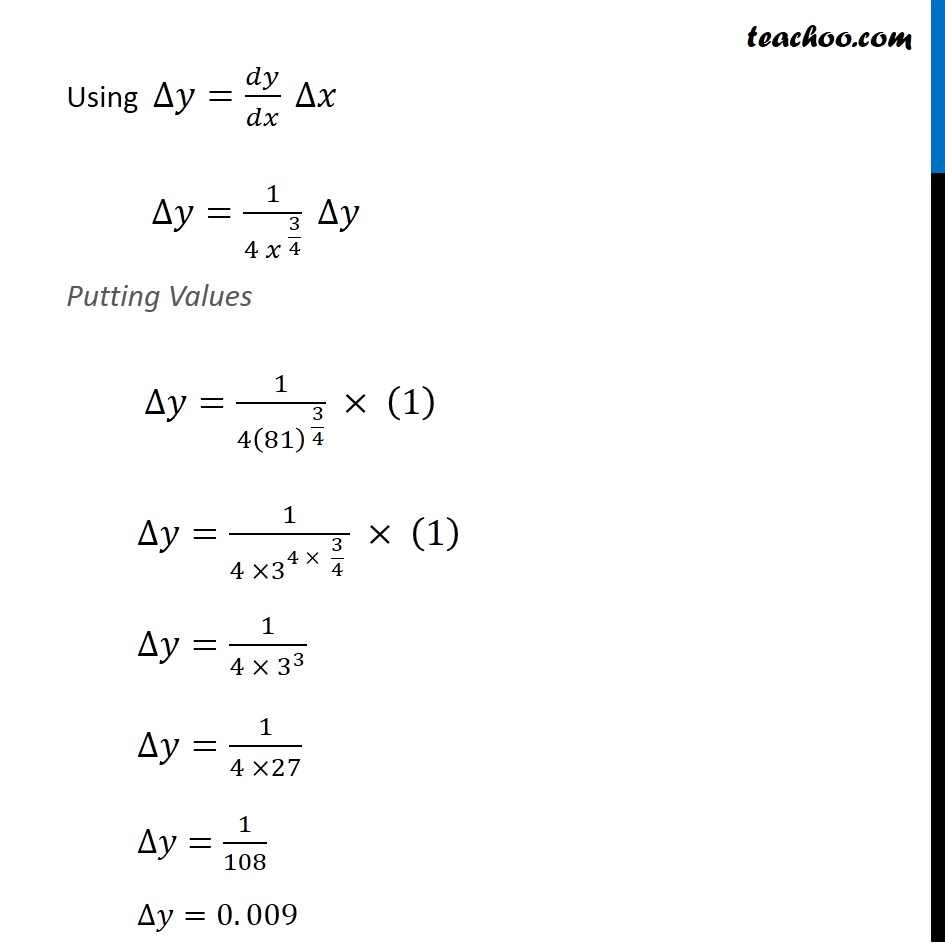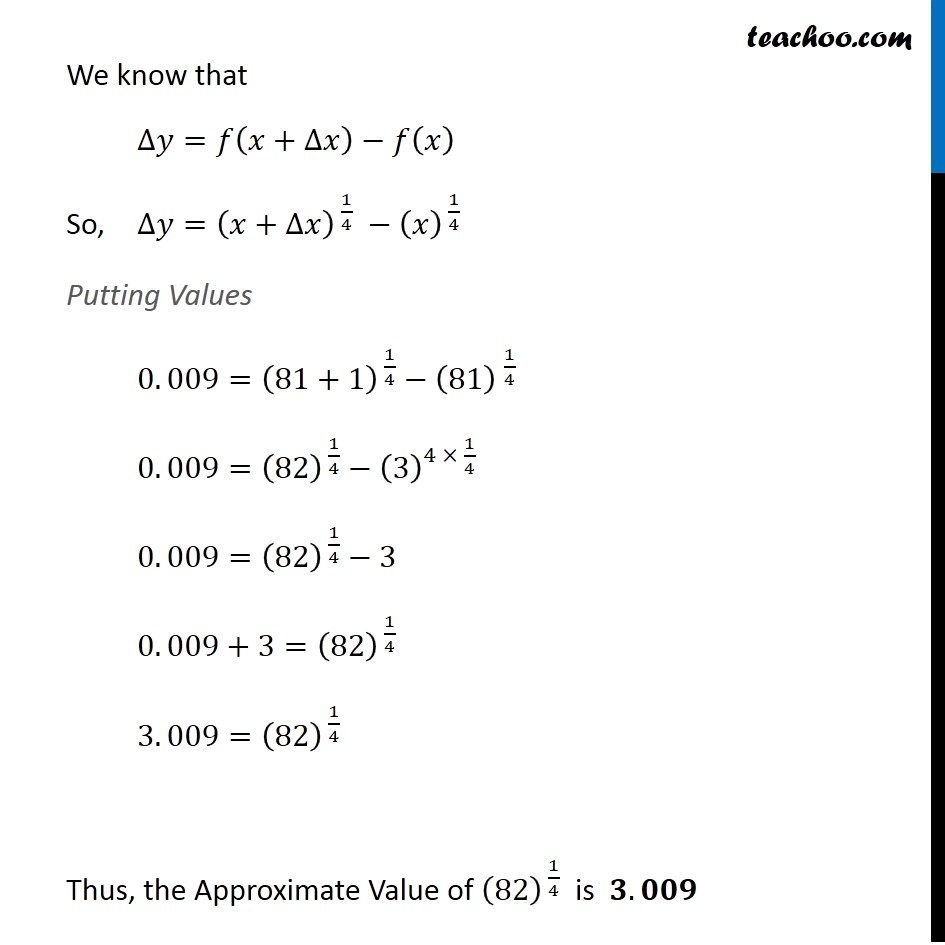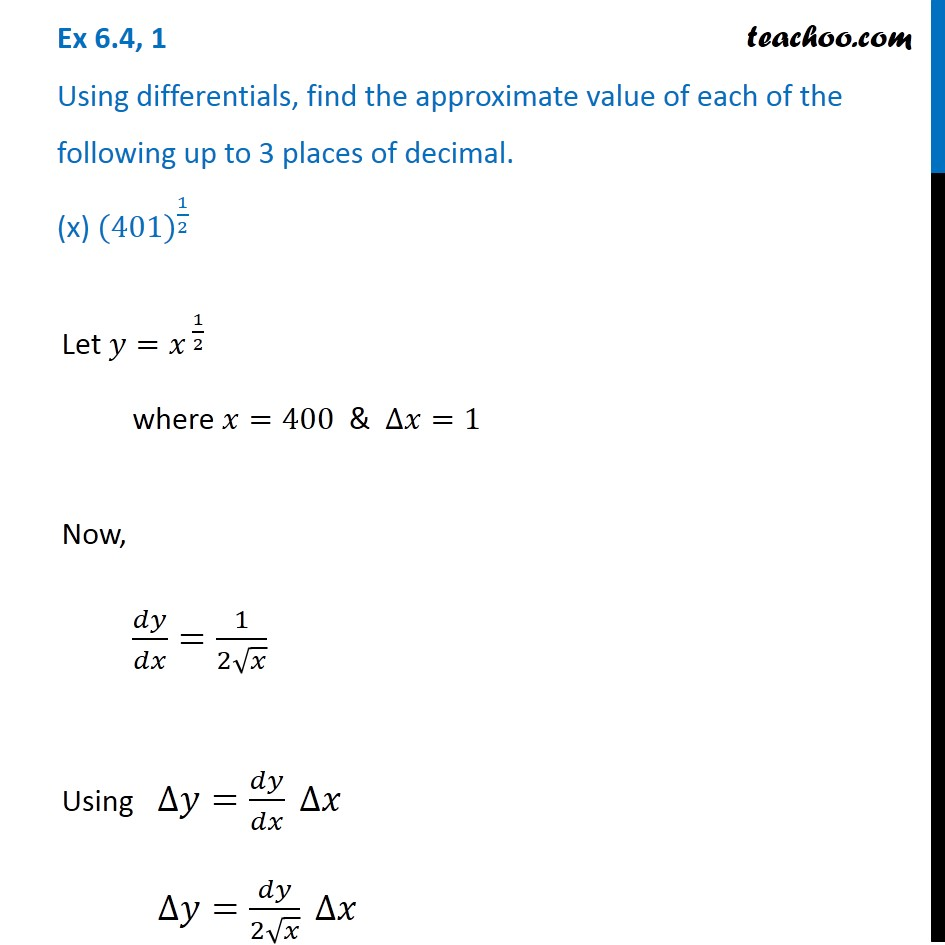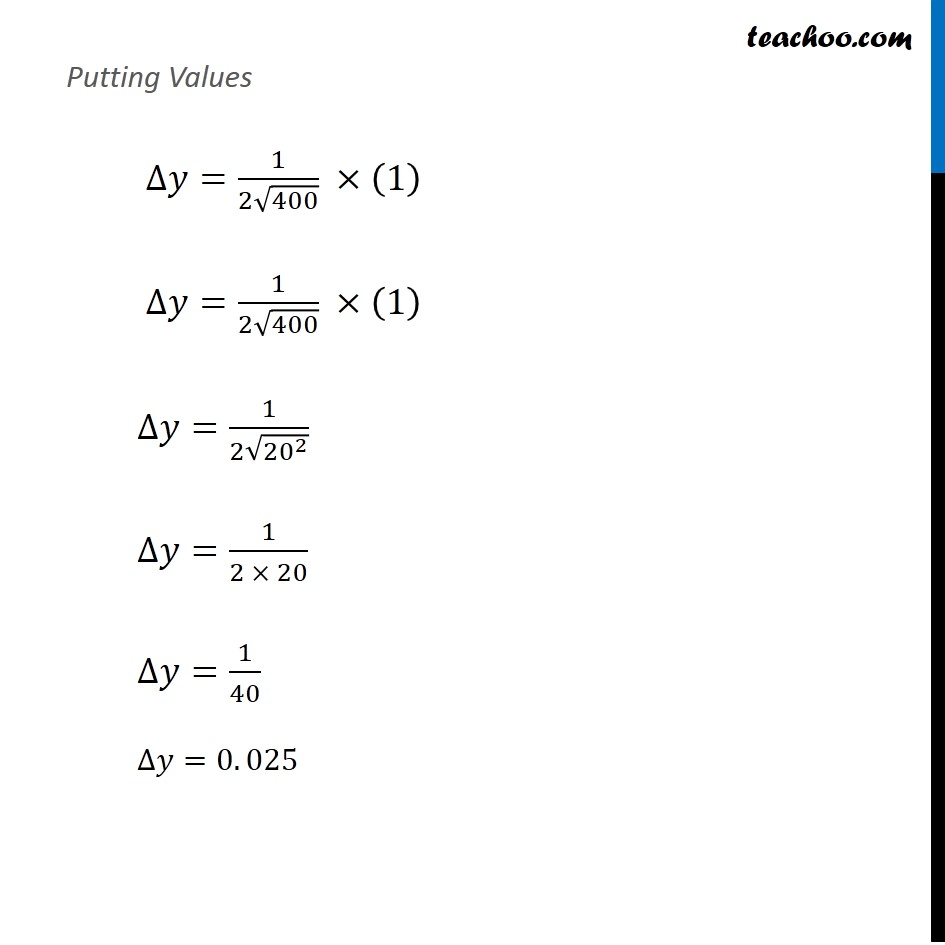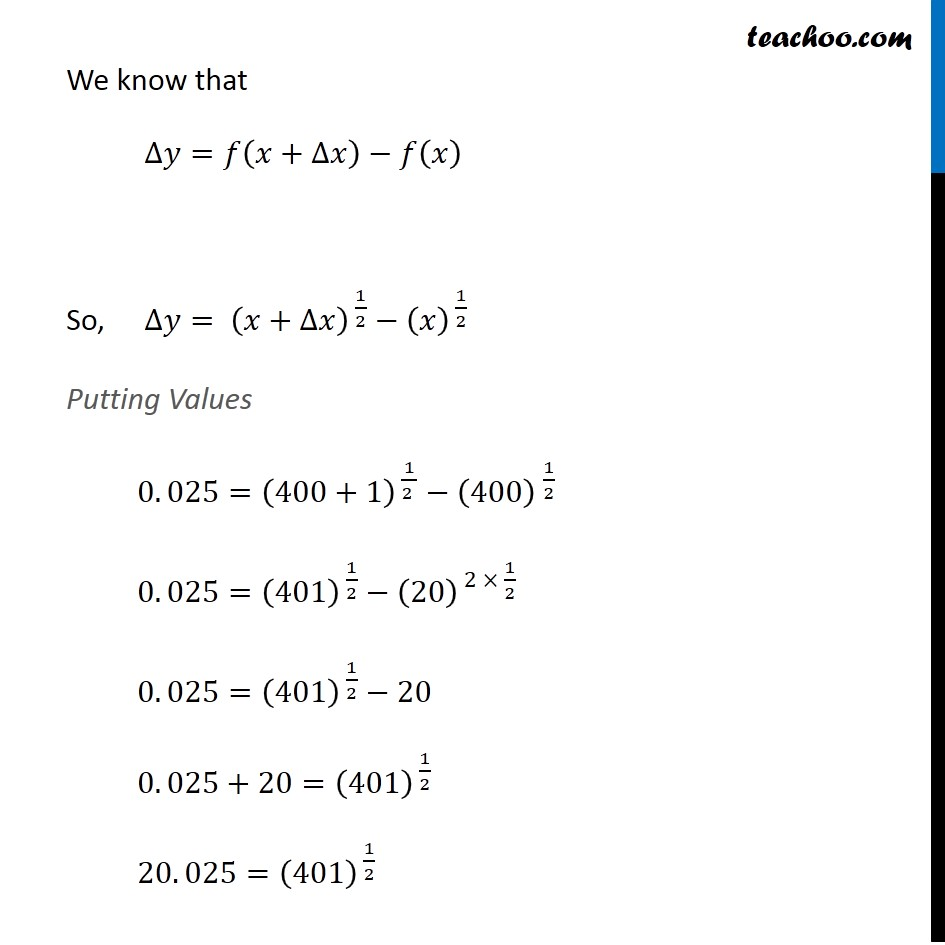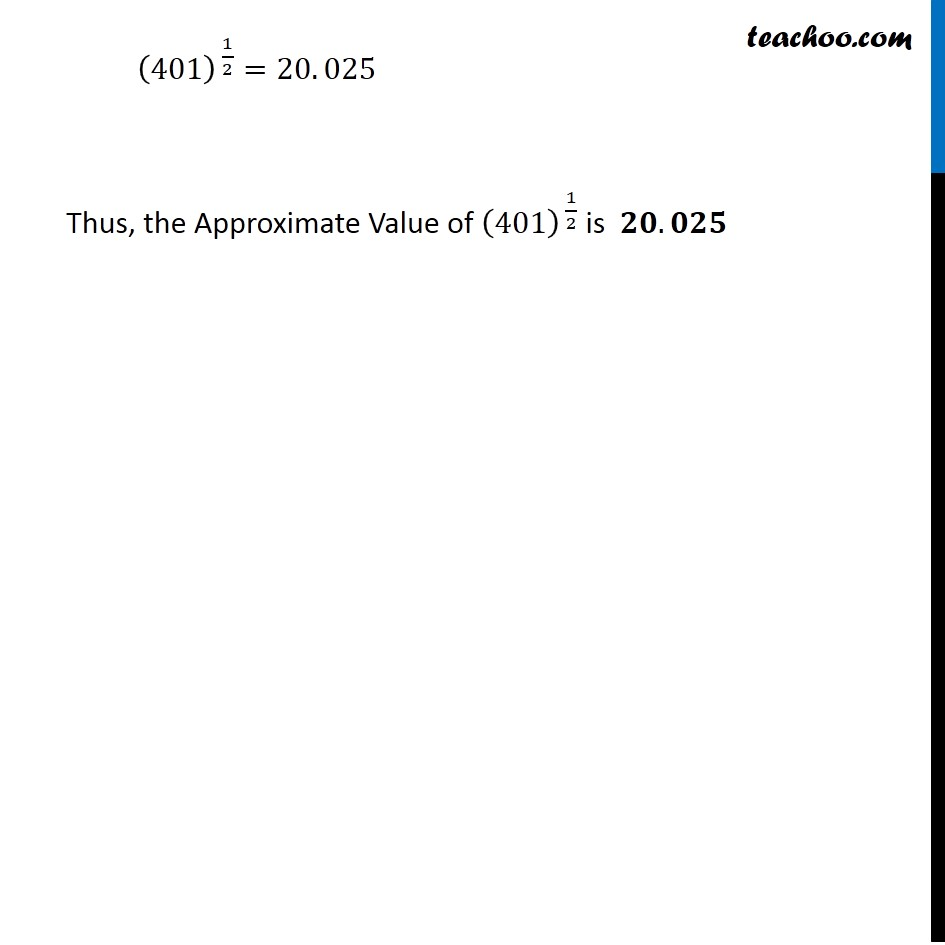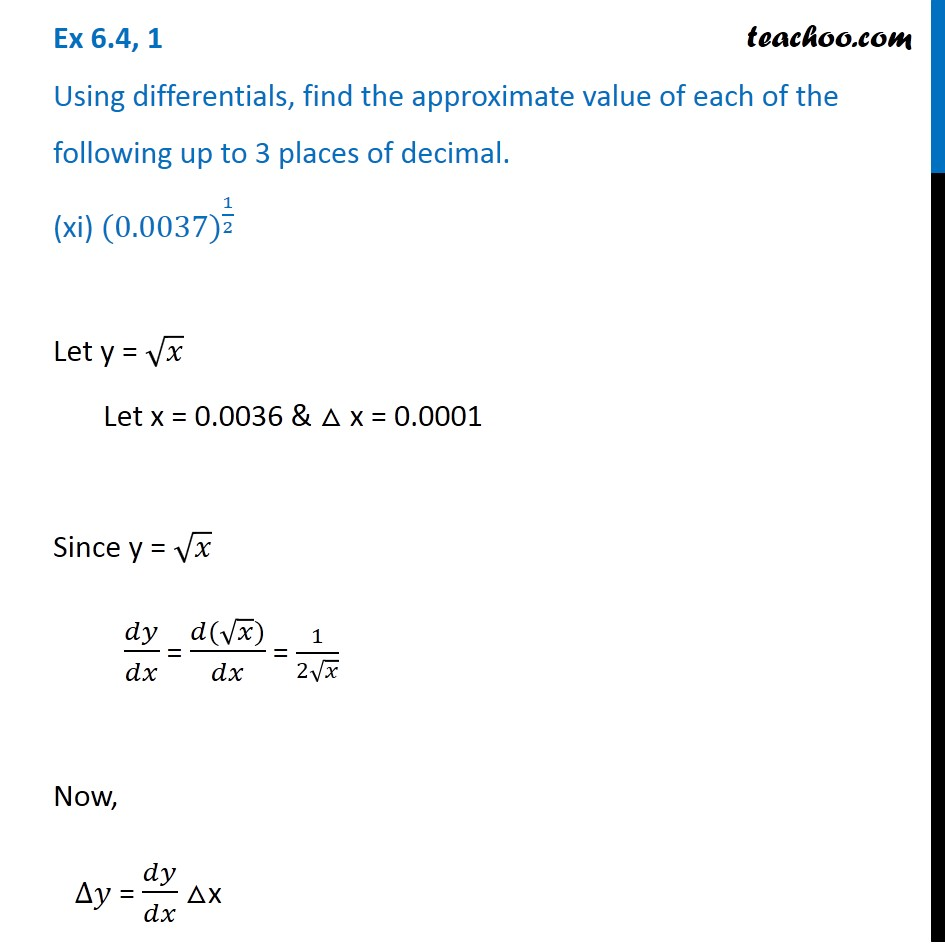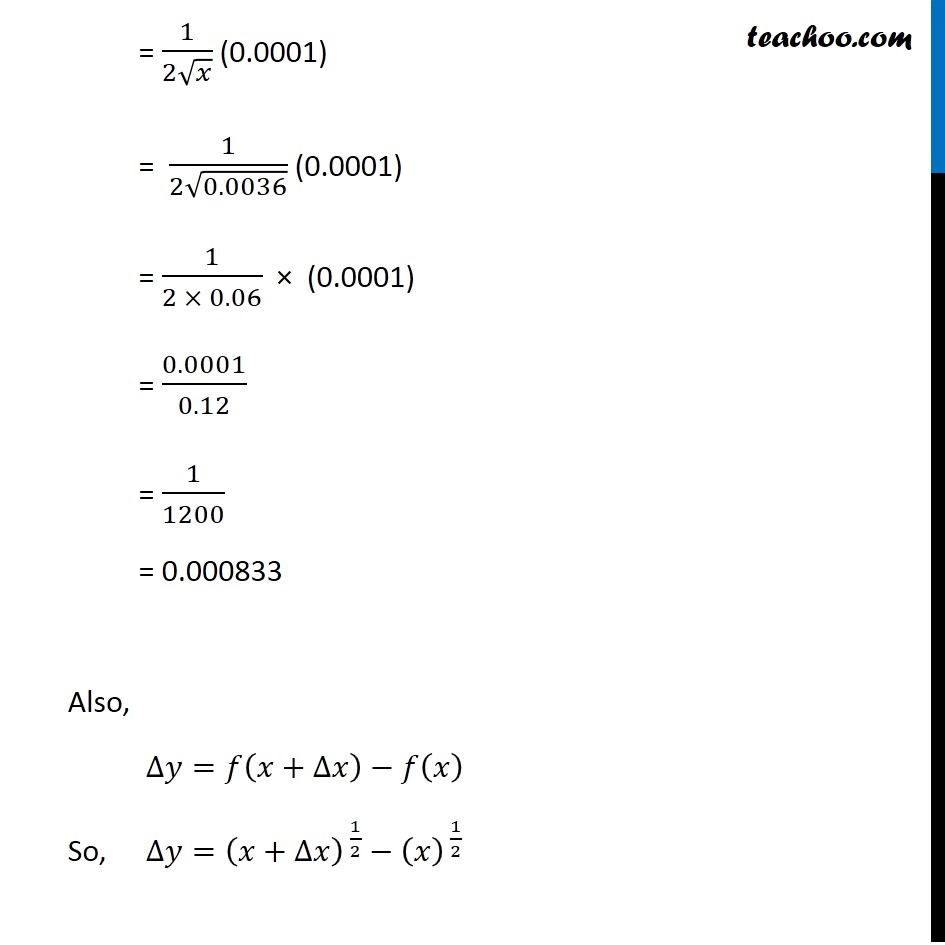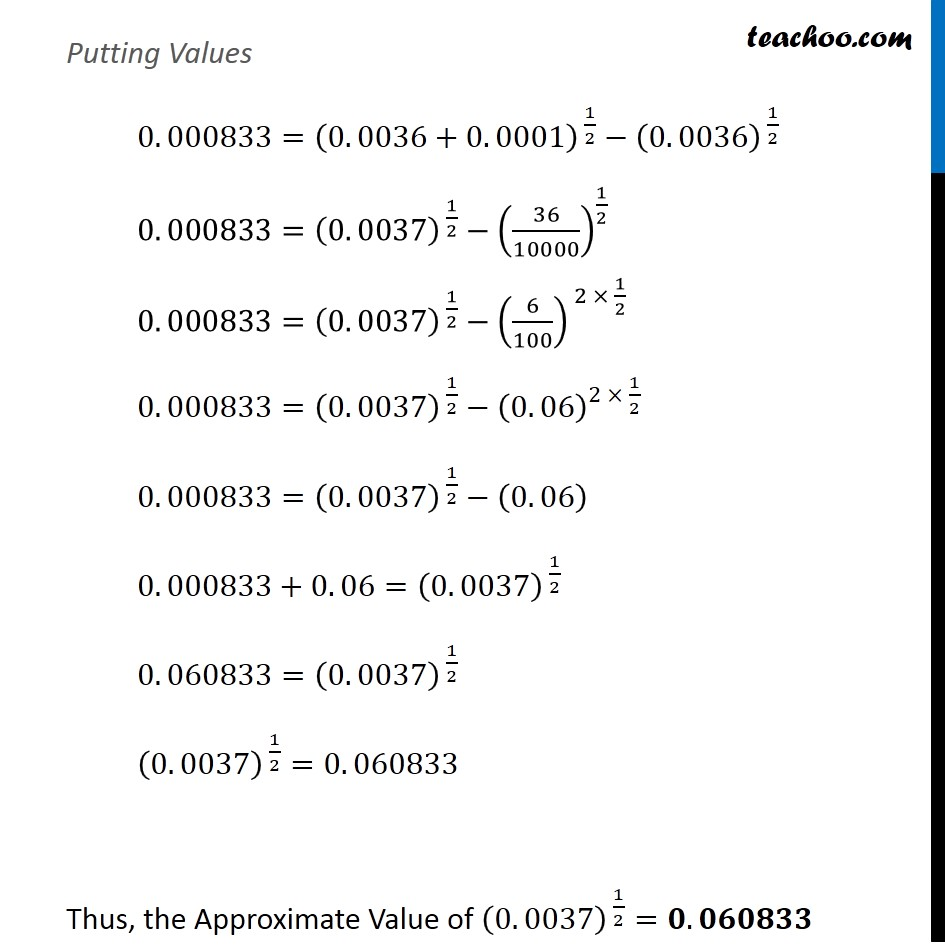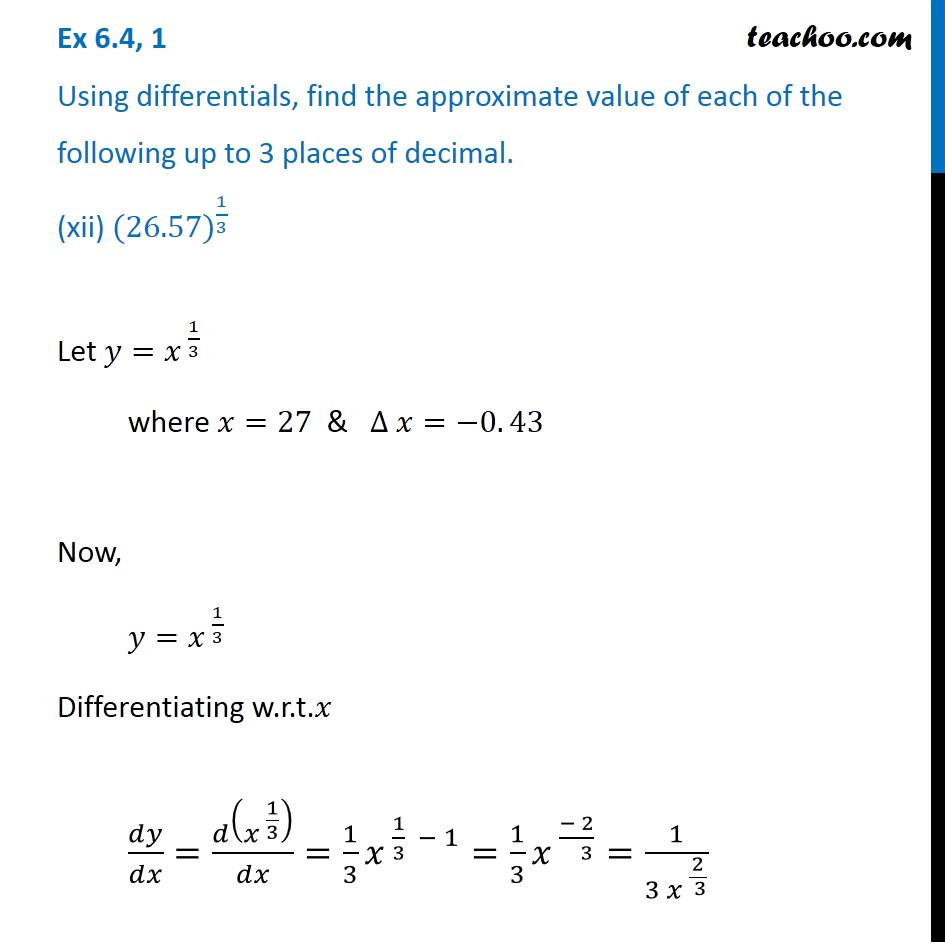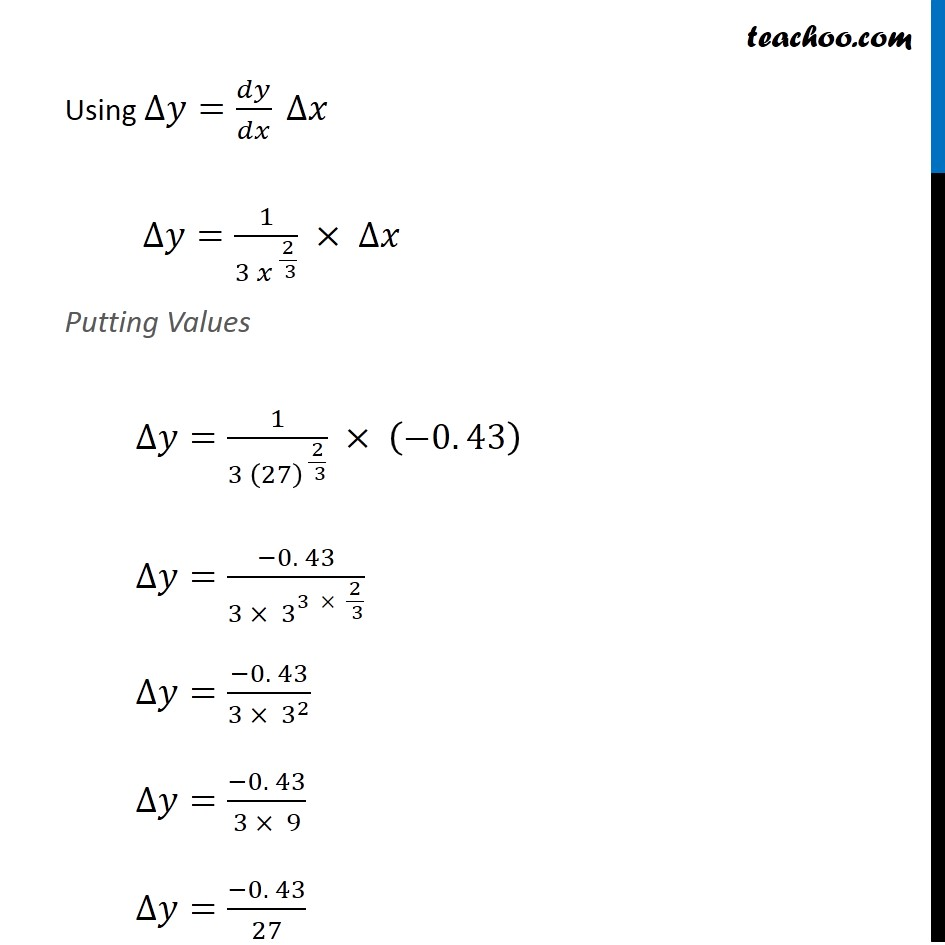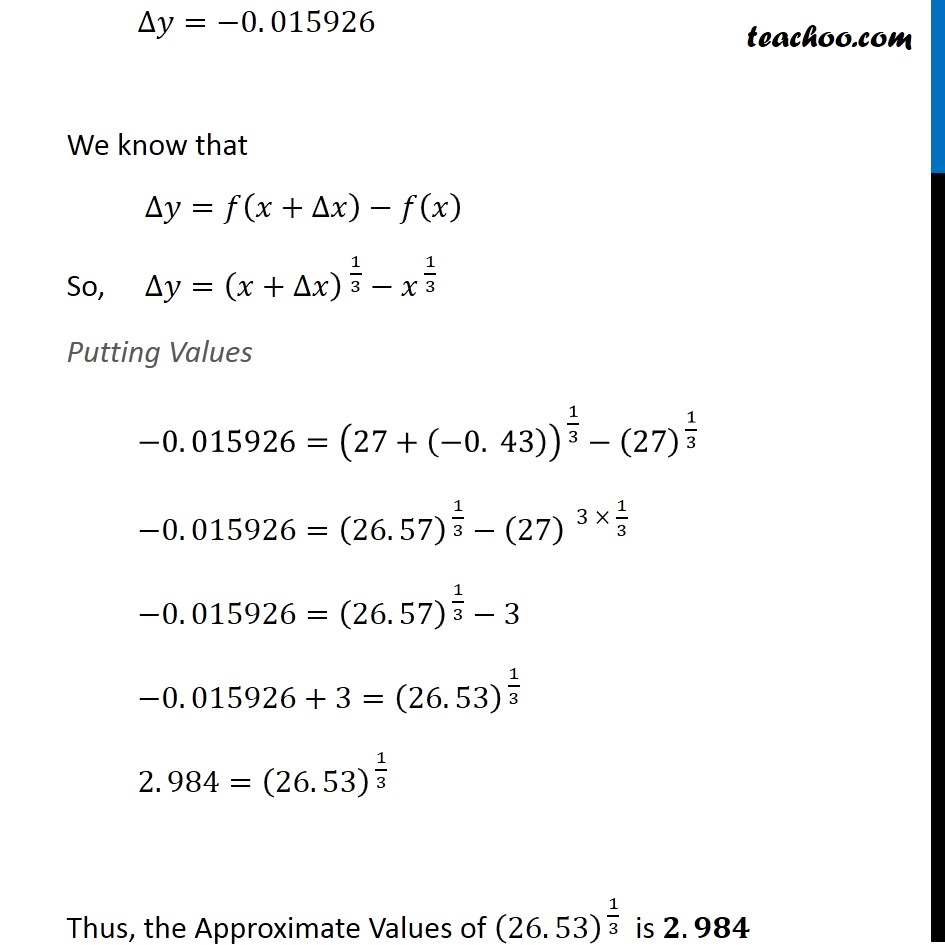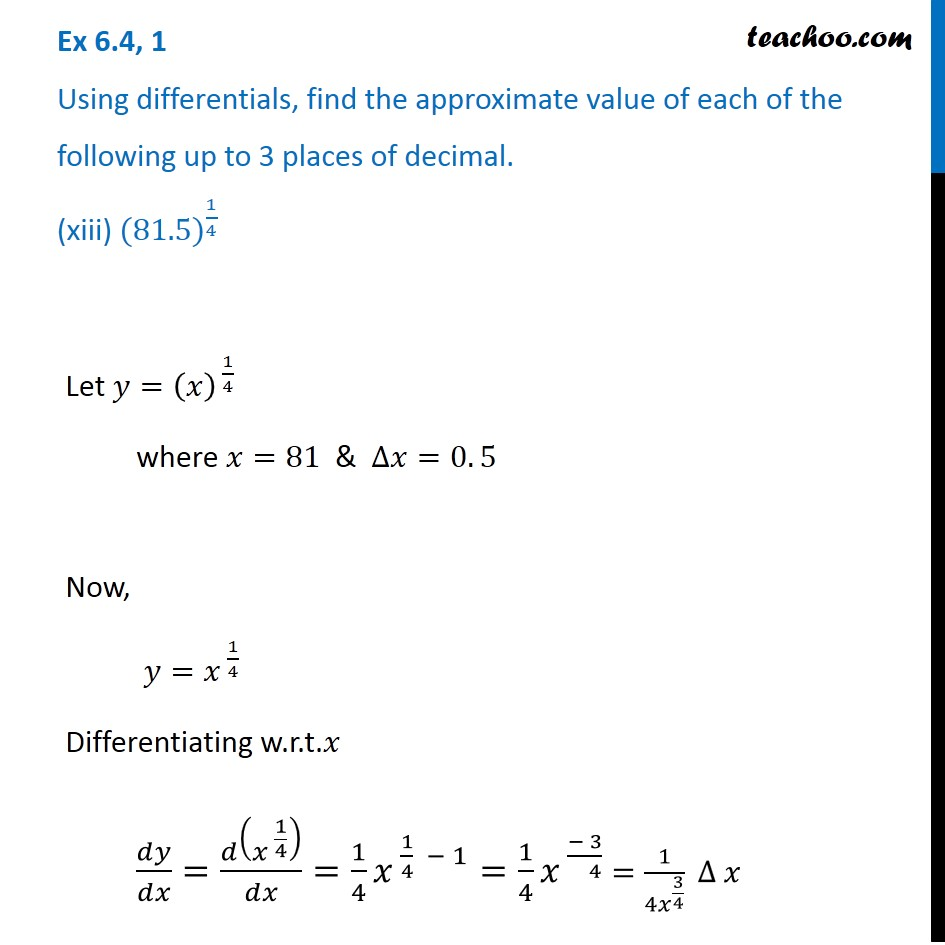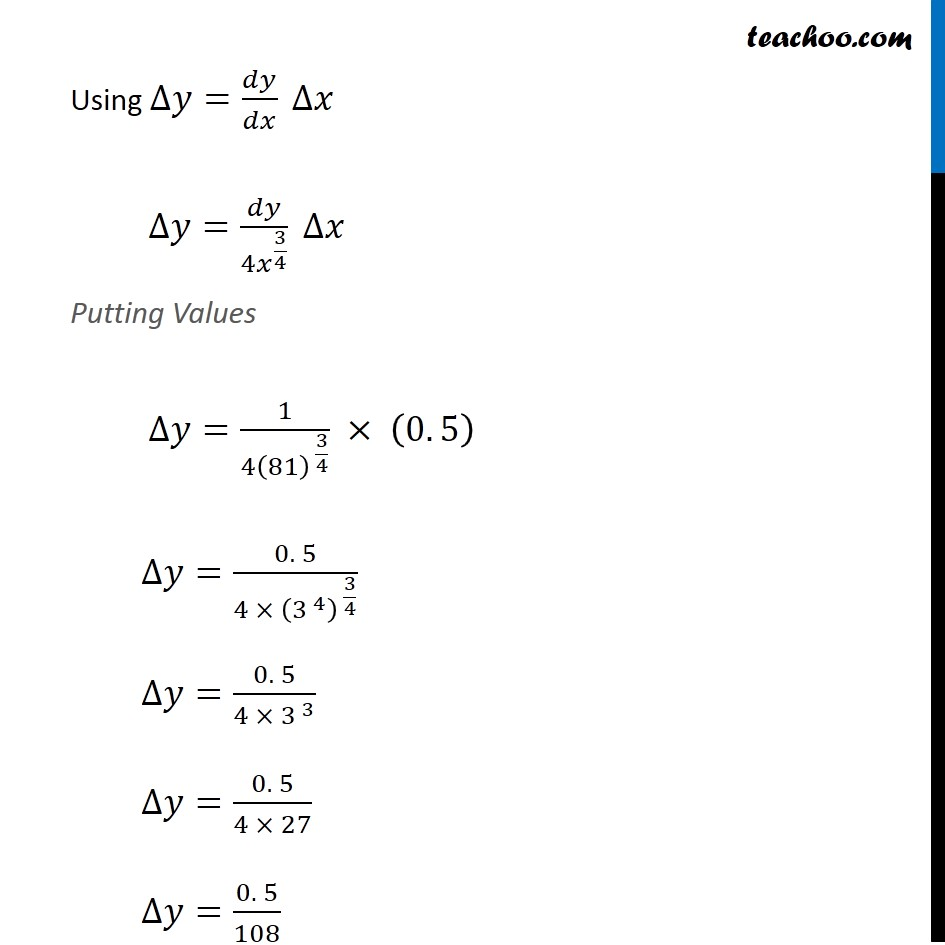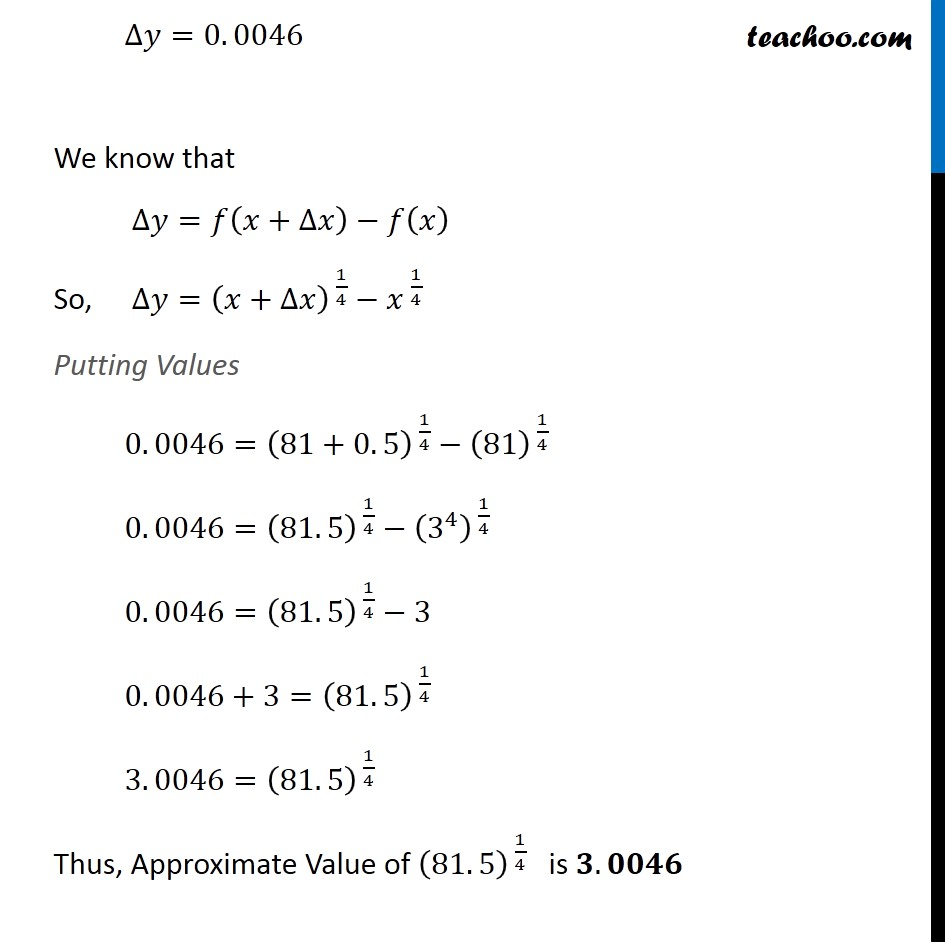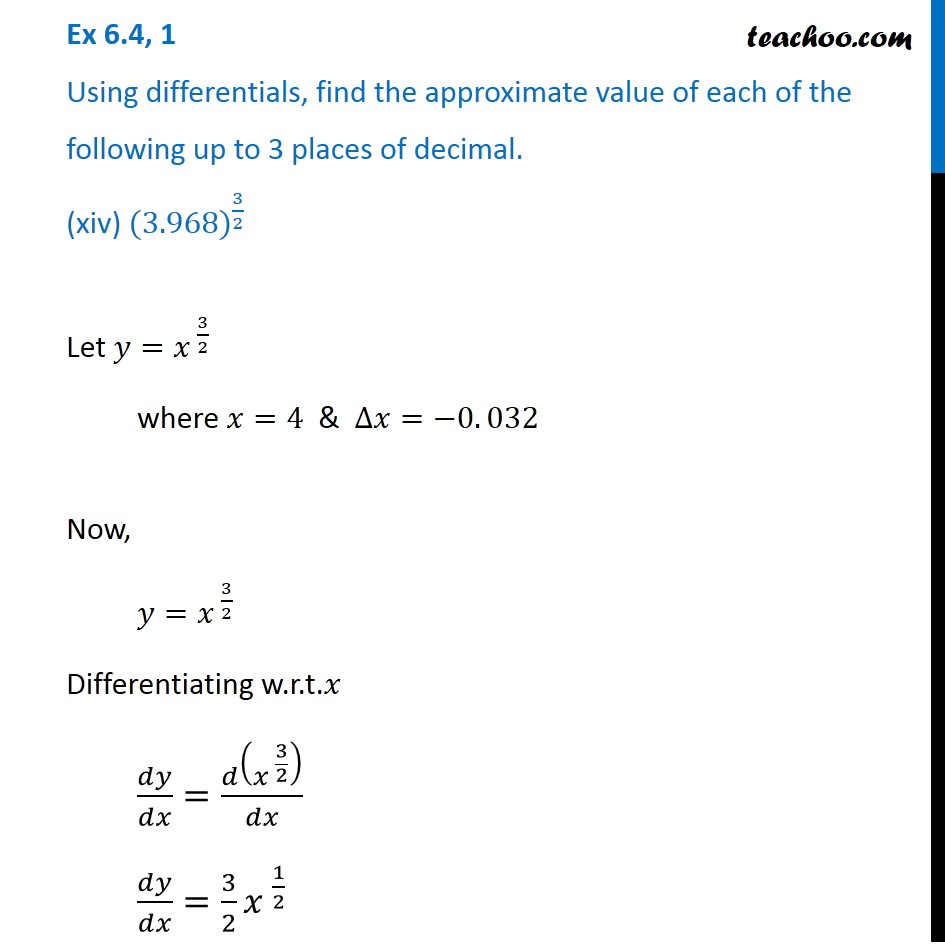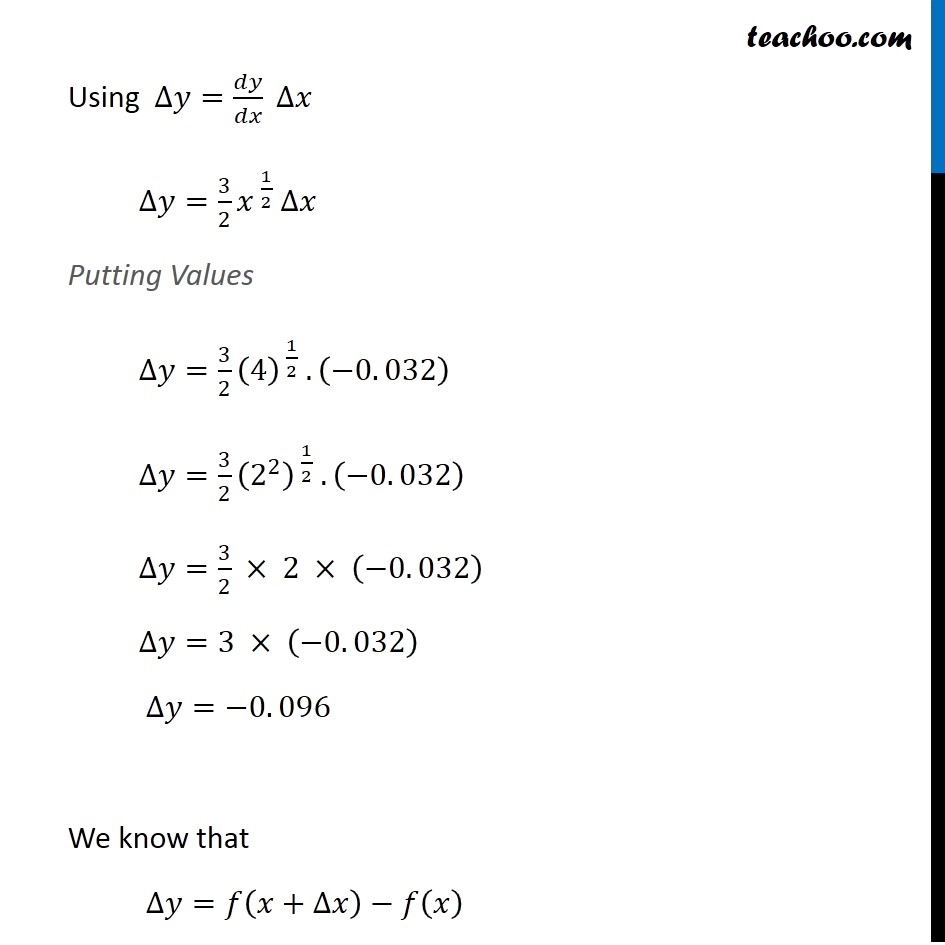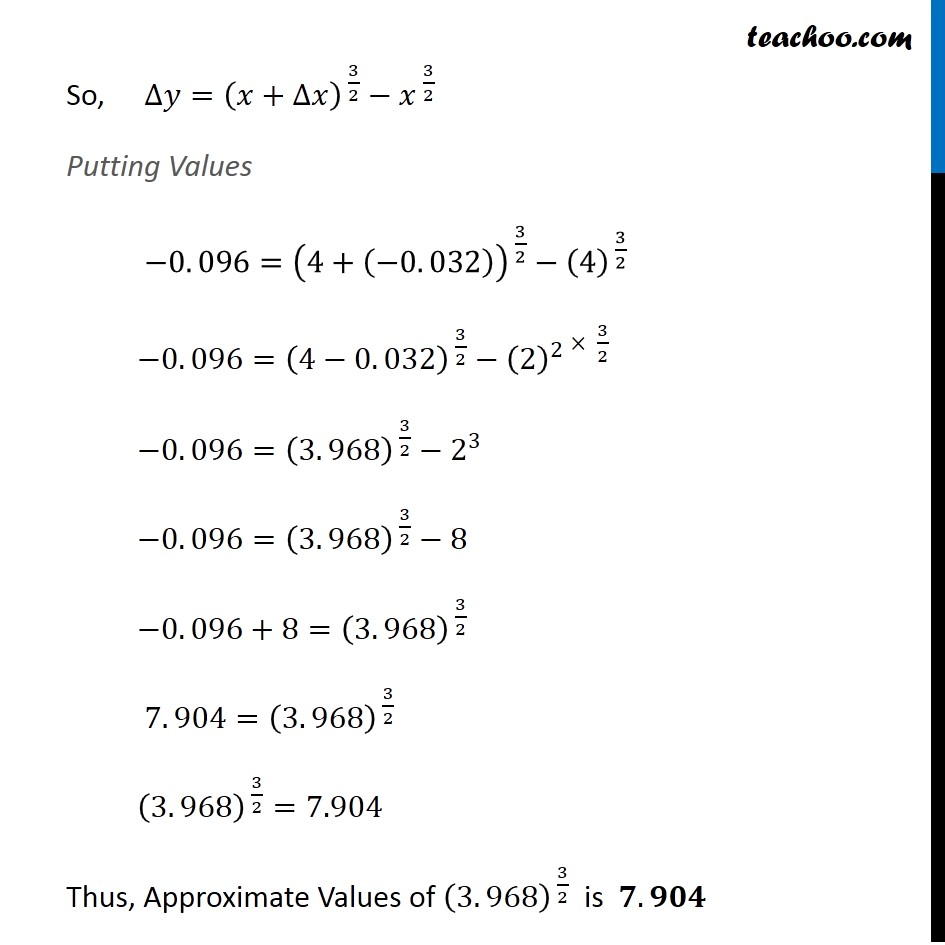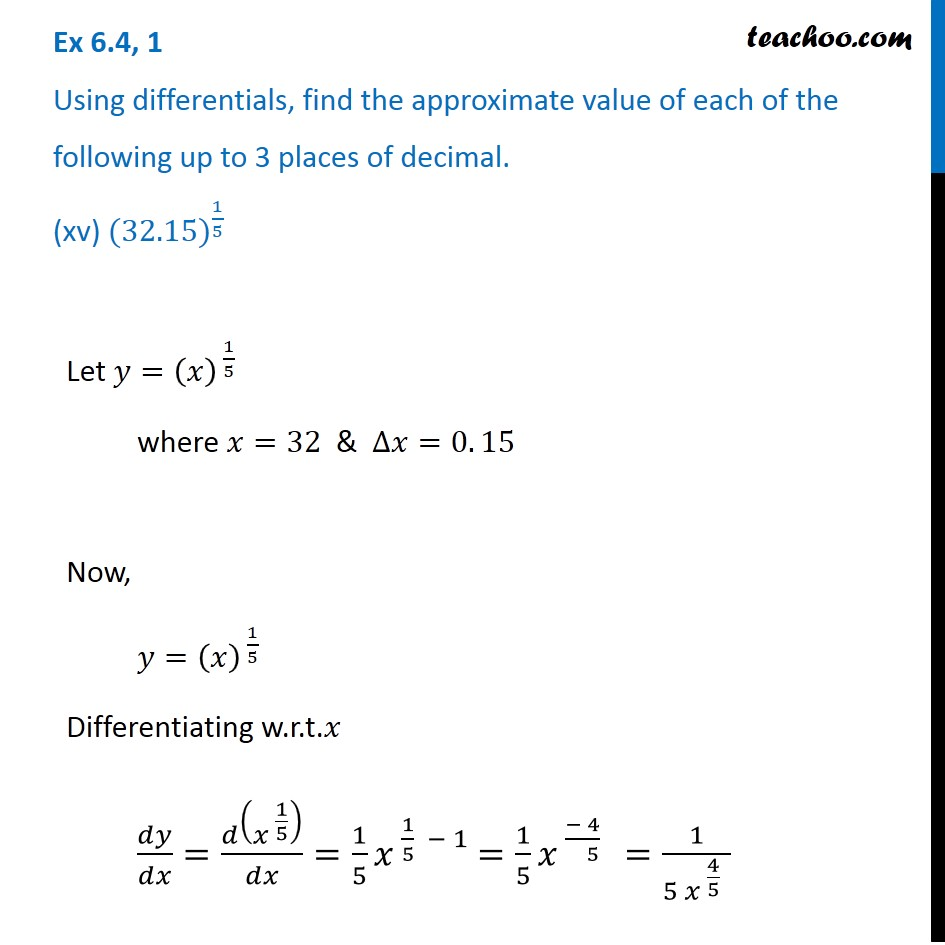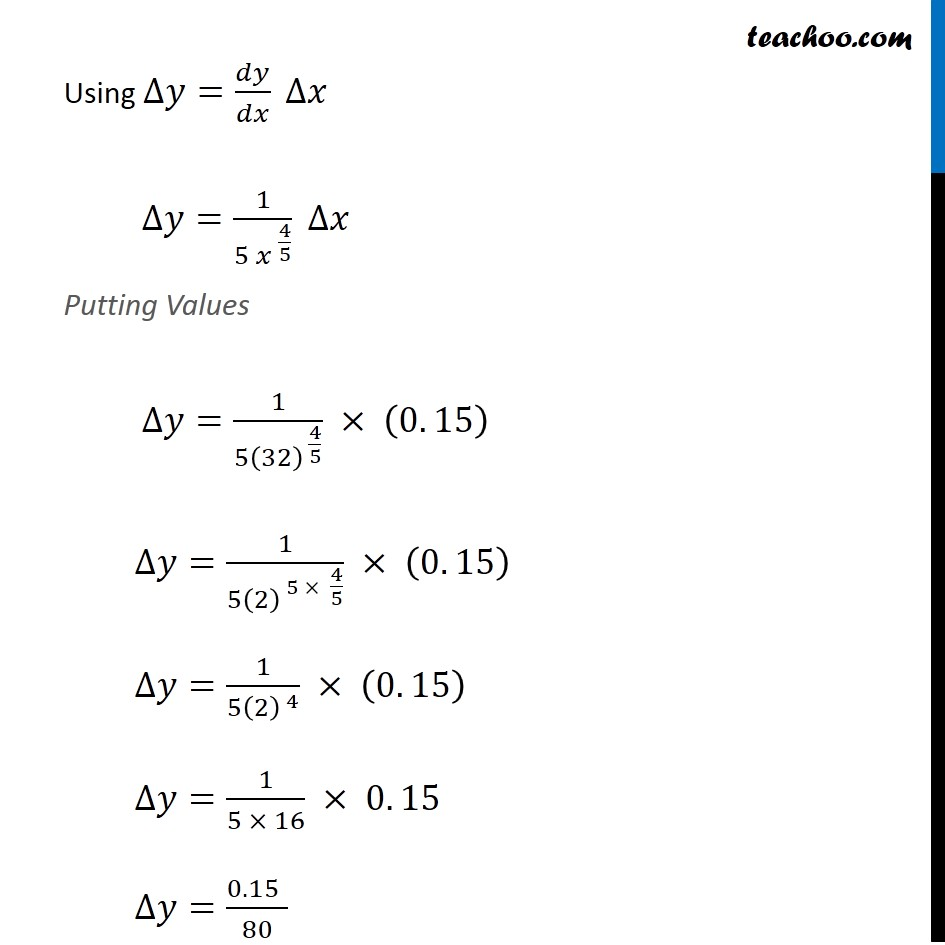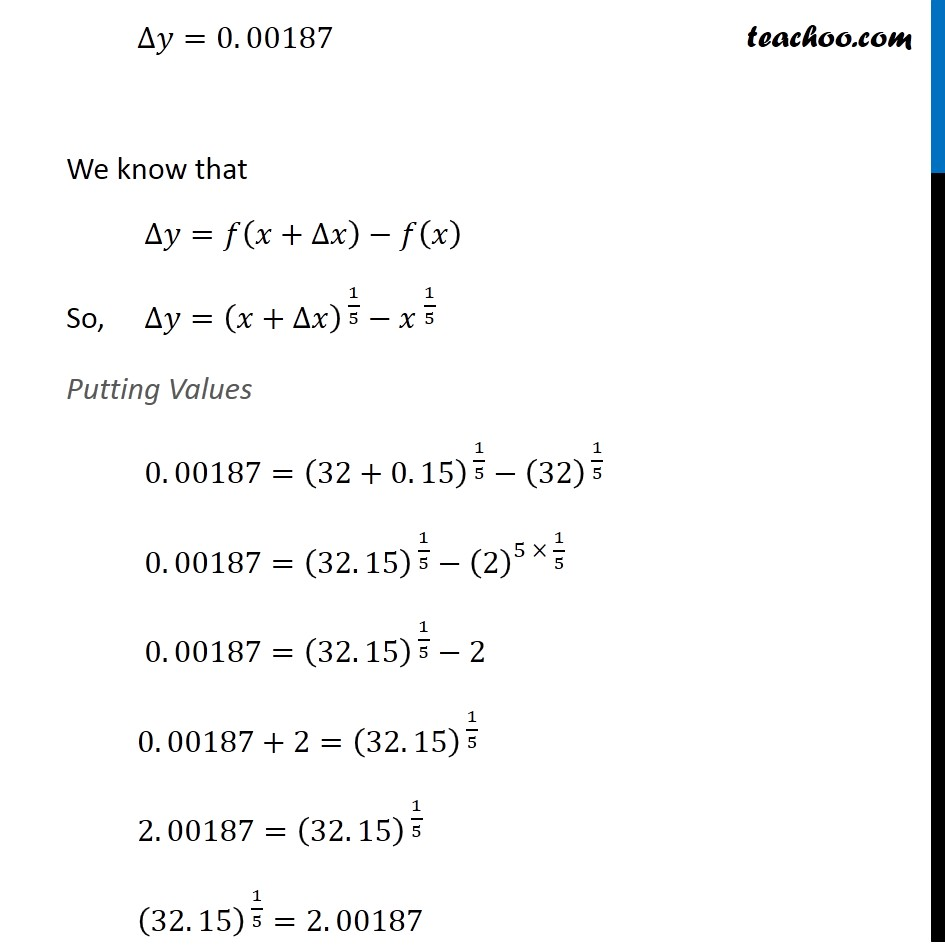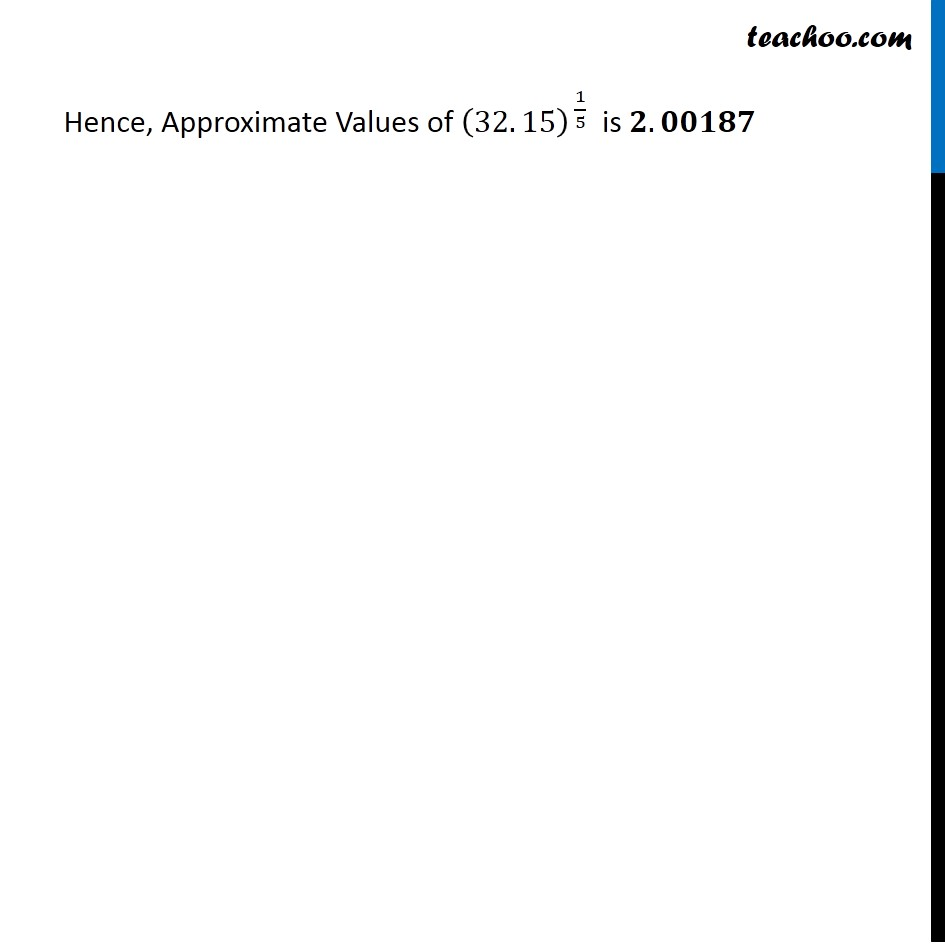1. Chapter 6 Class 12 Application of Derivatives
2. Serial order wise
3. Ex 6.4

Transcript

Ex 6.4, 1 Using differentials, find the approximate value of each of the following up to 3 places of decimal. (i) √25.3 Let y = √𝑥 where x = 25 & △ x = 0.3 Since y = √𝑥 𝑑𝑦/𝑑𝑥 = (𝑑(√𝑥))/𝑑𝑥 = 1/(2√𝑥) Now, ∆𝑦 = 𝑑𝑦/𝑑𝑥 △x = 1/(2√𝑥) (0.3) = 1/(2√25) (0.3) = 1/(2 × 5) × 0.3 = 0.3/10 = 0.03 Also, ∆𝑦=𝑓(𝑥+∆𝑥)−𝑓(𝑥) Putting values ∆𝑦=√(𝑥+∆𝑥)−√𝑥 0. 03=√(25+0. 3)−√25 0. 03=√(25. 3)−5 0. 03+5=√(25. 3) √(25. 3)=5. 03 Hence, approximate value of √25.3 is 5.03 Ex 6.4, 1 Using differentials, find the approximate value of each of the following up to 3 places of decimal. (ii) √49.5 Let y = √𝑥 where x = 49 & △ x = 0.5 Since y = √𝑥 𝑑𝑦/𝑑𝑥 = (𝑑(√𝑥))/𝑑𝑥 = 1/(2√𝑥) Now, ∆𝑦 = 𝑑𝑦/𝑑𝑥 △x = 1/(2√𝑥) (0.5) = 1/(2 × √49) × 0.5 = 1/(2 × 7) × 0.5 = 0.5/14 = 0.036 Also, ∆𝑦=𝑓(𝑥+∆𝑥)−𝑓(𝑥) Putting values ∆𝑦=√(𝑥+∆𝑥)−√𝑥 0. 036=√(49+0. 5)−√49 0. 036=√49.5−7 0. 036+7=√49.5 √49.5=7. 036 Hence, approximate value of √49.5 is 7.036 Ex 6.4, 1 Using differentials, find the approximate value of each of the following up to 3 places of decimal. (iii) √0.6 Let y = √𝑥 where x = 0.64 & △x = –0.04 Since y = √𝑥 𝑑𝑦/𝑑𝑥 = (𝑑(√𝑥))/𝑑𝑥 = 1/(2√𝑥) Now, ∆𝑦 = 𝑑𝑦/𝑑𝑥 △x = 1/(2√0.64) (–0.04) = 1/(2√(64/100)) (–0.04) = 1/(2 ×8/10) × (–0.04) = (−10 × 0.04)/16 = (−10 × 4)/(16 × 100) = –0.025 Also, ∆𝑦=𝑓(𝑥+∆𝑥)−𝑓(𝑥) Putting values ∆𝑦=√(𝑥+∆𝑥)−√𝑥 −0. 025=√(0.64−0.04)−√0.64 −0.025=√0.60−0.8 0.8 – 0. 025=√0.60 √0.60=0.775 Hence, approximate value of √0.60 is 0.775 Ex 6.4, 1 Using differentials, find the approximate value of each of the following up to 3 places of decimal. (iv) 〖(0.009)〗^(1/3) Let 𝑦=(𝑥)^(1/3) where 𝑥=0. 008 & ∆𝑥=0. 001 Differentiating w.r.t.𝑥 𝑑𝑦/𝑑𝑥=𝑑(〖𝑥 〗^(1/3) )/𝑑𝑥=1/3 𝑥^((−2)/3)=1/(3〖 𝑥〗^( 2/3) ) Using ∆𝑦=𝑑𝑦/𝑑𝑥 ∆𝑥 ∆𝑦=1/(3〖 𝑥〗^( 2/3) ) ×∆𝑥 Putting Values ∆𝑦=1/(3(0. 008)^( 2/3) ) ×0. 001 ∆𝑦=(0. 001)/(3(8/1000)^(2/3) ) ∆𝑦=(0. 001)/(3(2/10)^( 3 × 2/3) ) ∆𝑦=(0. 001)/(3(2/10)^2 ) ∆𝑦=(0. 001)/(3 × 4/100) ∆𝑦=(0.001 × 100)/12 ∆𝑦=0. 008 We know that ∆𝑦=𝑓(𝑥+∆𝑥)−𝑓(𝑥) ∆𝑦=〖(𝑥+∆𝑥) 〗^(1/3)−〖𝑥 〗^(1/3) Putting Values 0. 008=(0. 008" " +0. 001)^( 1/3)−(0. 008" " )^( 1/3) 0. 008=(0. 009)^( 1/3)−(0. 008)^( 1/3) 0. 008=(0. 009)^( 1/3)−(8/1000)^( 1/3) 0. 008=(0. 009)^( 1/3)−(2/10)^( 3 × 1/3) 0. 008=(0. 009)^( 1/3)−(2/10) 0. 008=(0. 009)^( 1/3)−0. 2 0. 008+0. 2=(0. 009)^( 1/3) (0. 009)^( 1/3)=0. 208 Thus , Approximate Value of (0 . 009)^(1/3) is 𝟎. 𝟐𝟎𝟖 Ex 6.4, 1 Using differentials, find the approximate value of each of the following up to 3 places of decimal. (v) 〖(0.999)〗^(1/10) Let 𝑦=〖𝑥 〗^(1/10) where 𝑥=1 , ∆𝑥=−0. 001 Now, 𝑦=𝑥^( 1/10) Differentiating w.r.t.𝑥 𝑑𝑦/𝑑𝑥=𝑑(𝑥^( 1/10) )/𝑑𝑥=1/10 𝑥^((−9)/10)=1/(10〖 𝑥〗^(9/10) ) Using ∆𝑦=𝑑𝑦/𝑑𝑥 ∆𝑥 Putting Values ∆𝑦= 1/(10〖 𝑥〗^(9/10) ) ∆𝑥 ∆𝑦= 1/(10 (1)^(9/10) ) × (−0. 001) ∆𝑦= 1/10 ×(−0. 001) ∆𝑦=−0. 0001 We know that ∆𝑦=𝑓(𝑥+∆𝑥)−𝑓(𝑥) ∆𝑦=〖(𝑥+∆𝑥) 〗^(1/10)−𝑥^( 1/10) Putting Values ∆𝑦=(1+(−0. 001))^(1/10)−(1)^(1/10) −0. 0001=(0. 999)^(1/10)−1 −0. 0001+1=(0. 999)^(1/10) 0. 9999=(0. 999)^(1/10) Thus, the Approximate Value of (0. 999)^(1/10) is 𝟎. 𝟗𝟗𝟗𝟗 Ex 6.4, 1 Using differentials, find the approximate value of each of the following up to 3 places of decimal. (vi) 〖(15)〗^(1/4)Let 𝑦=〖𝑥 〗^(1/4) where 𝑥=16 , ∆𝑥=−1 Now, 𝑦=𝑥^( 1/4) Differentiating w.r.t.𝑥 𝑑𝑦/𝑑𝑥=𝑑(𝑥^( 1/4) )/𝑑𝑥=1/4 𝑥^( (1 − 4)/4 )=1/4 𝑥^( (−3)/( 4) ) Let 𝑦=〖𝑥 〗^(1/4) where 𝑥=16 , ∆𝑥=−1 Now, 𝑦=𝑥^( 1/4) Differentiating w.r.t.𝑥 𝑑𝑦/𝑑𝑥=𝑑(𝑥^( 1/4) )/𝑑𝑥=1/4 𝑥^( (1 − 4)/4 )=1/4 𝑥^( (−3)/( 4) ) Using ∆𝑦=𝑑𝑦/𝑑𝑥 ∆𝑥 ∆𝑦=1/(4(𝑥)^( 3/4) ) ∆𝑥 Putting Values ∆𝑦=1/(4(16)^( 3/4) ) . (−1) ∆𝑦=1/(4(2^4 )^( 3/4) ) (−1) ∆𝑦=(−1)/(4 × 2^3 ) ∆𝑦=(−1)/(4 × 8) ∆𝑦=(−1)/32 ∆𝑦=−0. 03125 We know that ∆𝑦=𝑓(𝑥+∆𝑥)−𝑓(𝑥) ∆𝑦=(𝑥+∆𝑥)^(1/4)−(𝑥)^(1/4) Putting Values −0. 03125=(16+(−1))^( 1/4)−〖(16) 〗^(1/4) −0. 03125=(16−1)^( 1/4)−(2)^(4 × 1/4) −0. 03125=(15)^( 1/4)−2 −0. 03125+2=(15)^( 1/4) −0. 03125+2=(15)^( 1/4) 1. 96875=(15)^( 1/4) Thus, Approximate Value of (15)^( 1/4) is 𝟏. 𝟗𝟔𝟖𝟕𝟓 Ex 6.4, 1 Using differentials, find the approximate value of each of the following up to 3 places of decimal. (vii) 〖(26)〗^(1/3) Let 𝑦=(𝑥)^(1/3) where 𝑥=27 & ∆𝑥=−1 Now, 𝑦=〖𝑥 〗^(1/3) Differentiating w.r.t.𝑥 𝑑𝑦/𝑑𝑥=𝑑(〖𝑥 〗^(1/3) )/𝑑𝑥=1/3 〖𝑥 〗^(1/3 − 1) =1/3 〖𝑥 〗^((− 2)/( 3) )=1/(3〖𝑥 〗^(2/( 3) ) ) Using ∆𝑦=𝑑𝑦/𝑑𝑥 ∆𝑥 ∆𝑦=1/(3〖𝑥 〗^(2/( 3) ) ) ∆𝑥 Putting Values ∆𝑦=1/(3(27)^( 2/3 ) )× (−1) ∆𝑦=1/(3(3^3 )^( 2/3 ) )× (−1) ∆𝑦=(−1)/(3〖 × 3〗^(2 ) ) ∆𝑦=(−1)/(3 × 9 ) ∆𝑦=(−1)/27 ∆𝑦=−0. 037037 We know that ∆𝑦=𝑓(𝑥+∆𝑥)−𝑓(𝑥) ∆𝑦=〖(𝑥+∆𝑥) 〗^(1/3)−(𝑥)^( 1/3) Putting Values −0. 037037=〖(27+(−1)) 〗^(1/3)−(27)^( 1/3) −0. 037037=〖(26) 〗^(1/3)−(3)^( 3 × 1/3) −0. 037037=〖(26) 〗^(1/3)−3 −0. 037037+3=〖(26) 〗^(1/3) 2. 9629=〖(26) 〗^(1/3) Thus, Approximate Value of (26)^(1/3) is 𝟐. 𝟗𝟔𝟐𝟗 Ex 6.4, 1 Using differentials, find the approximate value of each of the following up to 3 places of decimal. (viii) 〖(255)〗^(1/4) Let 𝑦=(𝑥)^(1/4) where 𝑥=256 & ∆𝑥=−1 Now, 𝑦=(𝑥)^(1/4) Differentiating w.r.t.𝑥 𝑑𝑦/𝑑𝑥=𝑑(〖𝑥 〗^(1/4) )/𝑑𝑥=1/4 𝑥^( 1/4 − 1) =1/4 𝑥^( (−3)/( 4))=1/(4 𝑥^( 3/( 4)) ) Using ∆𝑦=𝑑𝑦/𝑑𝑥 ∆𝑥 ∆𝑦=1/(4〖 𝑥〗^( 3/( 4)) ) ×∆𝑥 Putting Values ∆𝑦=1/(4 (256)^(3/4) ) × (−1) ∆𝑦=(− 1)/(4 (4^4 )^(3/4) ) ∆𝑦=(− 1)/(4 × 4^3 ) ∆𝑦=(− 1)/(4 × 64) ∆𝑦=(− 1)/256 ∆𝑦=−0. 0039 We know that ∆𝑦=𝑓(𝑥+∆𝑥)−𝑓(𝑥) ∆𝑦=〖(𝑥+∆𝑥) 〗^(1/4)−𝑥^( 1/4) Putting Values −0. 0039=〖(256+(−1)) 〗^(1/4)−(256)^( 1/4) −0. 0039=〖(255) 〗^(1/4)−(4^4 )^( 1/4) −0. 0039=〖(255) 〗^(1/4)−4 −0. 0039=〖(255) 〗^(1/4)−4 −0. 0039+4=〖(255) 〗^(1/4) 3. 9961=〖(255) 〗^(1/4) Thus, the Approximate Values of 〖(255) 〗^(1/4) is 𝟑. 𝟗𝟗𝟔𝟏 Ex 6.4, 1 Using differentials, find the approximate value of each of the following up to 3 places of decimal. (ix) 〖(82)〗^(1/4) Let 𝑦=(𝑥)^( 1/4) where 𝑥=81 ∆𝑥=1 Now, 𝑦=(𝑥)^( 1/4) Differentiating w.r.t.𝑥 𝑑𝑦/𝑑𝑥=𝑑(𝑥^( 1/4) )/𝑑𝑥=1/4 〖𝑥 〗^(1/4 − 1)=1/4 〖𝑥 〗^((−3)/( 4))=1/(4〖𝑥 〗^(3/4) ) Using ∆𝑦=𝑑𝑦/𝑑𝑥 ∆𝑥 ∆𝑦=1/(4〖 𝑥 〗^(3/4) ) ∆𝑦 Putting Values ∆𝑦=1/(4(81)^( 3/4) ) × (1) ∆𝑦=1/(4 ×3^(4 × 3/4 ) ) × (1) ∆𝑦=1/(4 × 3^3 ) ∆𝑦=1/(4 ×27) ∆𝑦=1/108 ∆𝑦=0. 009 We know that ∆𝑦=𝑓(𝑥+∆𝑥)−𝑓(𝑥) So, ∆𝑦=(𝑥+∆𝑥)^( 1/4) −(𝑥)^( 1/4) Putting Values 0. 009=(81+1)^( 1/4)−〖(81) 〗^(1/4) 0. 009=(82)^( 1/4)−(3)^(4 × 1/4 ) 0. 009=(82)^( 1/4)−3 0. 009+3=(82)^( 1/4) 3. 009=(82)^( 1/4) Thus, the Approximate Value of (82)^( 1/4) is 𝟑. 𝟎𝟎𝟗 Ex 6.4, 1 Using differentials, find the approximate value of each of the following up to 3 places of decimal. (x) 〖(401)〗^(1/2) Let 𝑦=𝑥^( 1/2) where 𝑥=400 & ∆𝑥=1 Now, 𝑑𝑦/𝑑𝑥=1/(2√𝑥) Using ∆𝑦=𝑑𝑦/𝑑𝑥 ∆𝑥 ∆𝑦=𝑑𝑦/(2√𝑥) ∆𝑥 Putting Values ∆𝑦=1/(2√400) ×(1) ∆𝑦=1/(2√400) ×(1) ∆𝑦=1/(2√(〖20〗^2 )) ∆𝑦=1/(2 × 20) ∆𝑦=1/40 ∆𝑦=0. 025 We know that ∆𝑦=𝑓(𝑥+∆𝑥)−𝑓(𝑥) So, ∆𝑦= (𝑥+∆𝑥)^( 1/2)−(𝑥)^( 1/2) Putting Values 0. 025=(400+1)^( 1/(2 ))−〖(400) 〗^(1/2) 0. 025=(401)^( 1/2)−(20)^( 2 × 1/2) 0. 025=(401)^( 1/2)−20 0. 025+20=(401)^( 1/2) 20. 025=(401)^( 1/2) (401)^( 1/2)=20. 025 Thus, the Approximate Value of (401)^( 1/2) is 𝟐𝟎. 𝟎𝟐𝟓 Ex 6.4, 1 Using differentials, find the approximate value of each of the following up to 3 places of decimal. (xi) 〖(0.0037)〗^(1/2) Let y = √𝑥 Let x = 0.0036 & △ x = 0.0001 Since y = √𝑥 𝑑𝑦/𝑑𝑥 = (𝑑(√𝑥))/𝑑𝑥 = 1/(2√𝑥) Now, ∆𝑦 = 𝑑𝑦/𝑑𝑥 △x = 1/(2√𝑥) (0.0001) = 1/(2√0.0036) (0.0001) = 1/(2 × 0.06) × (0.0001) = 0.0001/0.12 = 1/1200 = 0.000833 Also, ∆𝑦=𝑓(𝑥+∆𝑥)−𝑓(𝑥) So, ∆𝑦=(𝑥+∆𝑥)^( 1/2)−(𝑥)^( 1/2) Putting Values 0. 000833=(0. 0036+0. 0001)^( 1/2)−(0. 0036)^( 1/2) 0. 000833=(0. 0037)^( 1/2)−(36/10000)^(1/2) 0. 000833=(0. 0037)^( 1/2)−(6/100)^( 2 × 1/2) 0. 000833=(0. 0037)^( 1/2)−(0. 06)^(2 × 1/2 ) 0. 000833=(0. 0037)^( 1/2)−(0. 06) 0. 000833+0. 06=(0. 0037)^( 1/2) 0. 060833=(0. 0037)^( 1/2) (0. 0037)^( 1/2)=0. 060833 Thus, the Approximate Value of (0. 0037)^( 1/2)=𝟎. 𝟎𝟔𝟎𝟖𝟑𝟑 Ex 6.4, 1 Using differentials, find the approximate value of each of the following up to 3 places of decimal. (xii) 〖(26.57)〗^(1/3) Let 𝑦=𝑥^( 1/3) where 𝑥=27 & ∆ 𝑥=−0. 43 Now, 𝑦=𝑥^( 1/3) Differentiating w.r.t.𝑥 𝑑𝑦/𝑑𝑥=𝑑(𝑥^( 1/3) )/𝑑𝑥=1/3 𝑥^( 1/3 − 1)=1/3 𝑥^( (− 2)/( 3))=1/(3 𝑥^( 2/( 3)) ) Using ∆𝑦=𝑑𝑦/𝑑𝑥 ∆𝑥 ∆𝑦=1/(3 𝑥^( 2/( 3)) ) × ∆𝑥 Putting Values ∆𝑦=1/(3 (27)^( 2/( 3)) ) × (−0. 43) ∆𝑦=(−0. 43)/(3 × 3^(3 × 2/( 3)) ) ∆𝑦=(−0. 43)/(3 × 3^2 ) ∆𝑦=(−0. 43)/(3 × 9) ∆𝑦=(−0. 43)/27 ∆𝑦=−0. 015926 We know that ∆𝑦=𝑓(𝑥+∆𝑥)−𝑓(𝑥) So, ∆𝑦=〖(𝑥+∆𝑥) 〗^(1/3)−𝑥^( 1/3) Putting Values −0. 015926=(27+(−0. 43))^( 1/3)−(27)^( 1/3) −0. 015926=(26. 57)^( 1/3)−(27)^( 3 × 1/3) −0. 015926=(26. 57)^( 1/3)−3 −0. 015926+3=(26. 53)^( 1/3) 2. 984=(26. 53)^( 1/3) Thus, the Approximate Values of (26. 53)^( 1/3) is 𝟐. 𝟗𝟖𝟒 Ex 6.4, 1 Using differentials, find the approximate value of each of the following up to 3 places of decimal. (xiii) 〖(81.5)〗^(1/4) Let 𝑦=(𝑥)^( 1/4) where 𝑥=81 & ∆𝑥=0. 5 Now, 𝑦=𝑥^( 1/4) Differentiating w.r.t.𝑥 𝑑𝑦/𝑑𝑥=𝑑(𝑥^( 1/4) )/𝑑𝑥=1/4 𝑥^( 1/4 − 1)=1/4 𝑥^( (− 3)/( 4)) =1/(4𝑥^(3/4) ) ∆ 𝑥 Using ∆𝑦=𝑑𝑦/𝑑𝑥 ∆𝑥 ∆𝑦=𝑑𝑦/(4𝑥^(3/4) ) ∆𝑥 Putting Values ∆𝑦=1/(4(81)^( 3/4) ) × (0. 5) ∆𝑦=(0. 5)/(4 × (3^( 4) )^( 3/4) ) ∆𝑦=(0. 5)/(4 × 3^( 3) ) ∆𝑦=(0. 5)/(4 × 27) ∆𝑦=(0. 5)/108 ∆𝑦=0. 0046 We know that ∆𝑦=𝑓(𝑥+∆𝑥)−𝑓(𝑥) So, ∆𝑦=(𝑥+∆𝑥)^( 1/4)−𝑥^( 1/4) Putting Values 0. 0046=(81+0. 5)^( 1/4)−(81)^( 1/4) 0. 0046=(81. 5)^( 1/4)−(3^4 )^( 1/4) 0. 0046=(81. 5)^( 1/4)−3 0. 0046+3=(81. 5)^( 1/4) 3. 0046=(81. 5)^( 1/4) Thus, Approximate Value of (81. 5)^( 1/4) is 𝟑. 𝟎𝟎𝟒𝟔 Ex 6.4, 1 Using differentials, find the approximate value of each of the following up to 3 places of decimal. (xiv) 〖(3.968)〗^(3/2) Let 𝑦=𝑥^( 3/2) where 𝑥=4 & ∆𝑥=−0. 032 Now, 𝑦=𝑥^( 3/2) Differentiating w.r.t.𝑥 𝑑𝑦/𝑑𝑥=𝑑(𝑥^( 3/2) )/𝑑𝑥 𝑑𝑦/𝑑𝑥=3/2 〖𝑥 〗^(1/2) Using ∆𝑦=𝑑𝑦/𝑑𝑥 ∆𝑥 ∆𝑦=3/2 𝑥^( 1/2) ∆𝑥 Putting Values ∆𝑦=3/2 (4)^( 1/2) . (−0. 032) ∆𝑦=3/2 (2^2 )^( 1/2) . (−0. 032) ∆𝑦=3/2 × 2 × (−0. 032) ∆𝑦=3 × (−0. 032) ∆𝑦=−0. 096 We know that ∆𝑦=𝑓(𝑥+∆𝑥)−𝑓(𝑥) So, ∆𝑦=(𝑥+∆𝑥)^( 3/2)−𝑥^( 3/2) Putting Values −0. 096=(4+(−0. 032))^( 3/2)−(4)^( 3/2) −0. 096=(4−0. 032)^( 3/2)−〖(2)^2〗^( × 3/2) −0. 096=(3. 968)^( 3/2)−2^3 −0. 096=(3. 968)^( 3/2)−8 −0. 096+8=(3. 968)^( 3/2) 7. 904=(3. 968)^( 3/2) (3. 968)^( 3/2)=7.904 Thus, Approximate Values of (3. 968)^( 3/2) is 𝟕. 𝟗𝟎𝟒 Ex 6.4, 1 Using differentials, find the approximate value of each of the following up to 3 places of decimal. (xv) 〖(32.15)〗^(1/5) Let 𝑦=(𝑥)^( 1/5) where 𝑥=32 & ∆𝑥=0. 15 Now, 𝑦=(𝑥)^( 1/5) Differentiating w.r.t.𝑥 𝑑𝑦/𝑑𝑥=𝑑(𝑥^( 1/5) )/𝑑𝑥=1/5 𝑥^( 1/5 − 1) =1/5 𝑥^( (− 4)/( 5) )=1/(5〖 𝑥〗^( 4/5 ) ) Using ∆𝑦=𝑑𝑦/𝑑𝑥 ∆𝑥 ∆𝑦=1/(5 〖𝑥 〗^(4/5) ) ∆𝑥 Putting Values ∆𝑦=1/(5(32)^( 4/5) ) × (0. 15) ∆𝑦=1/(5(2)^( 5 × 4/5) ) × (0. 15) ∆𝑦=1/(5(2)^( 4) ) × (0. 15) ∆𝑦=1/(5 × 16) × 0. 15 ∆𝑦=(0. 15" " )/80 ∆𝑦=0. 00187 We know that ∆𝑦=𝑓(𝑥+∆𝑥)−𝑓(𝑥) So, ∆𝑦=(𝑥+∆𝑥)^( 1/5)−〖𝑥 〗^(1/5) Putting Values 0. 00187=(32+0. 15)^( 1/5)−(32)^( 1/5) 0. 00187=(32. 15)^( 1/5)−(2)^(5 × 1/5 ) 0. 00187=(32. 15)^( 1/5)−2 0. 00187+2=(32. 15)^( 1/5) 2. 00187=(32. 15)^( 1/5) (32. 15)^( 1/5)=2. 00187 Hence, Approximate Values of (32. 15)^( 1/5) is 𝟐. 𝟎𝟎𝟏𝟖𝟕 Hence, Approximate Values of (32. 15)^( 1/5) is 𝟐. 𝟎𝟎𝟏𝟖𝟕

Ex 6.4